# RD Sharma Solutions for Class 10 Maths Chapter 13 Probability Exercise 13.1

The numerical value to the degree of uncertainty is known as probability. In this exercise students will be solving problems pertaining to games of chance such as tossing a coin, drawing a card from a pack of cards, throwing a die or dice. The RD Sharma Solutions Class 10 is a precious tool for students to clarify doubts and enhance the understanding of problems of their class. Students wishing to strengthen concepts of this exercise can also download the RD Sharma Solutions for Class 10 Maths Chapter 13 Probability Exercise 13.1 PDF provided below.

## RD Sharma Solutions for Class 10 Maths Chapter 13 Probability Exercise 13.1 Download PDF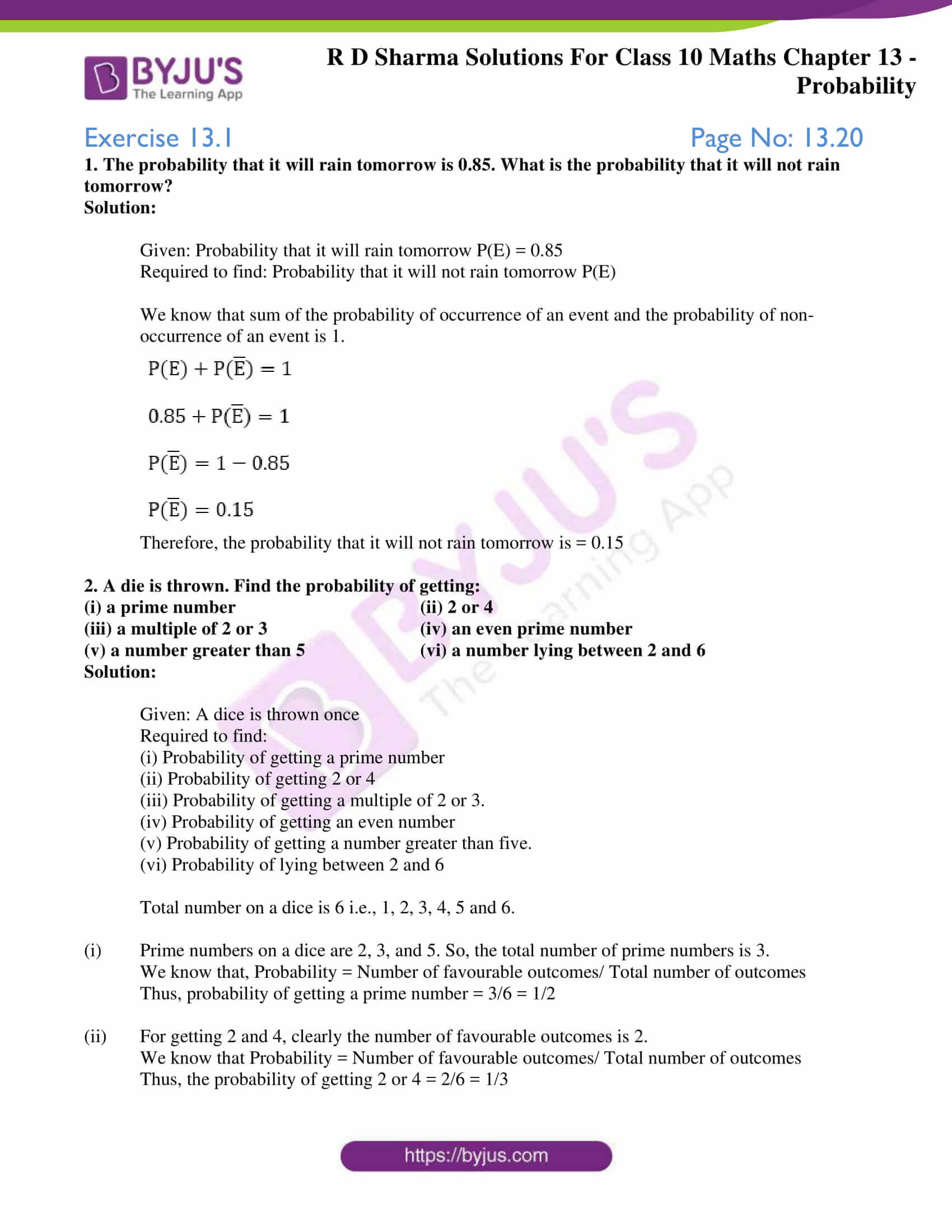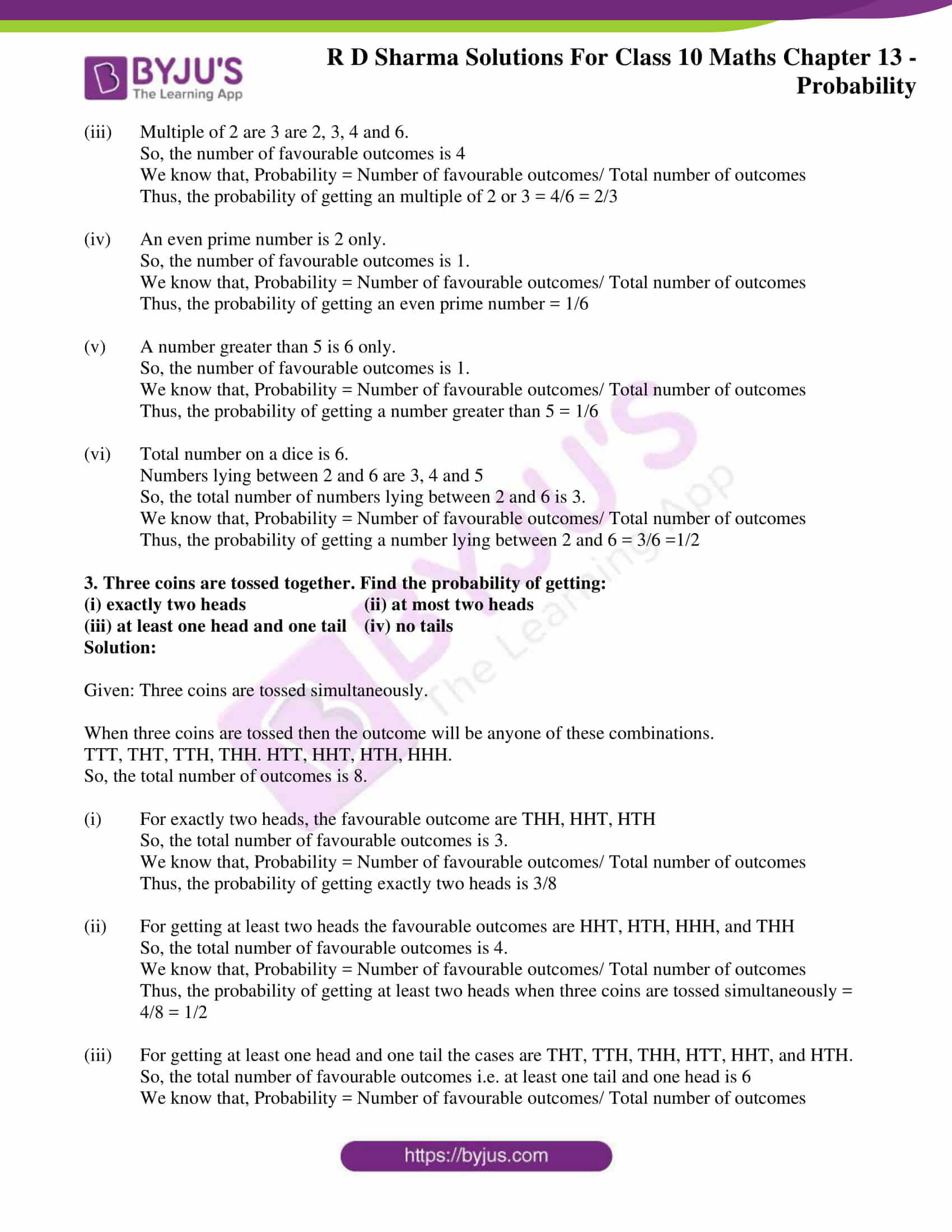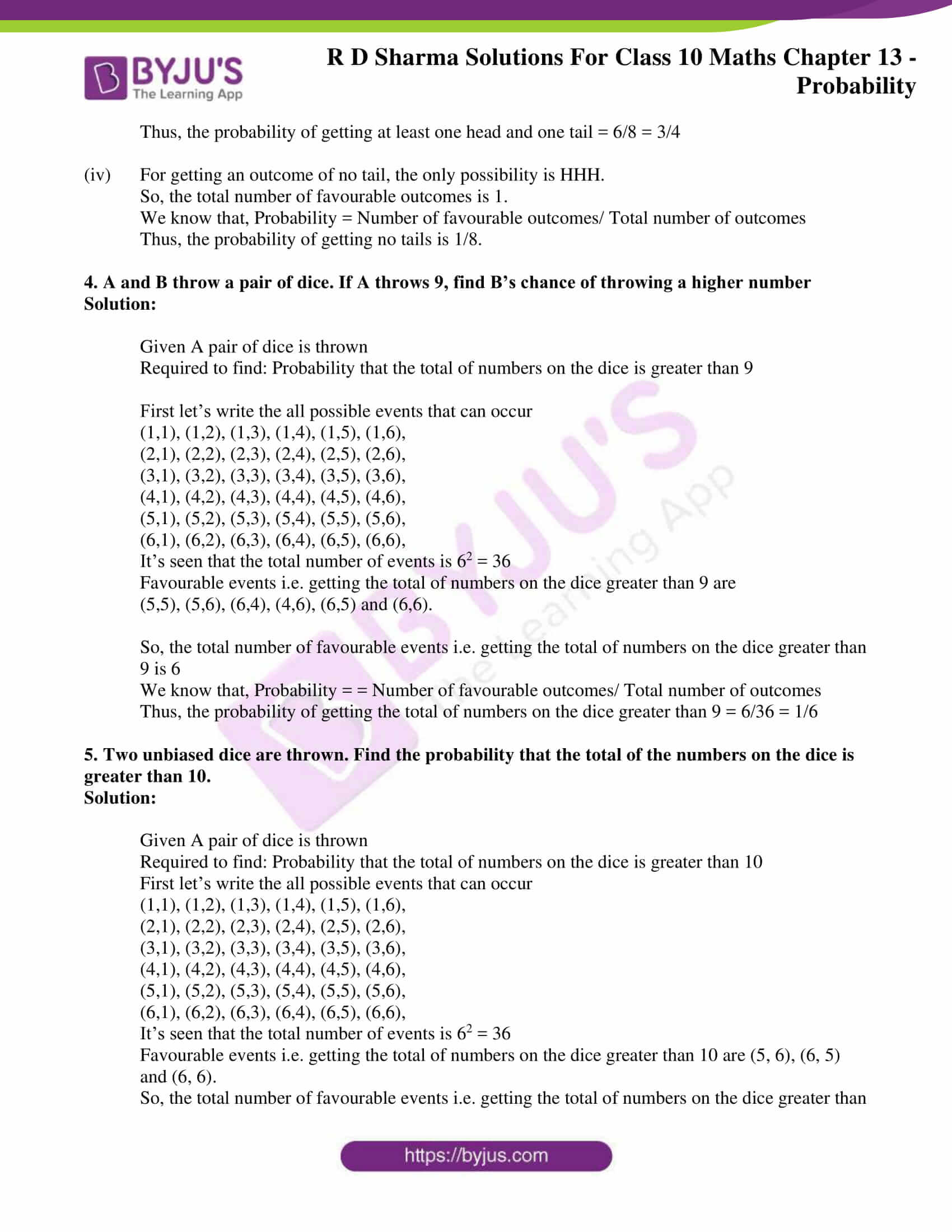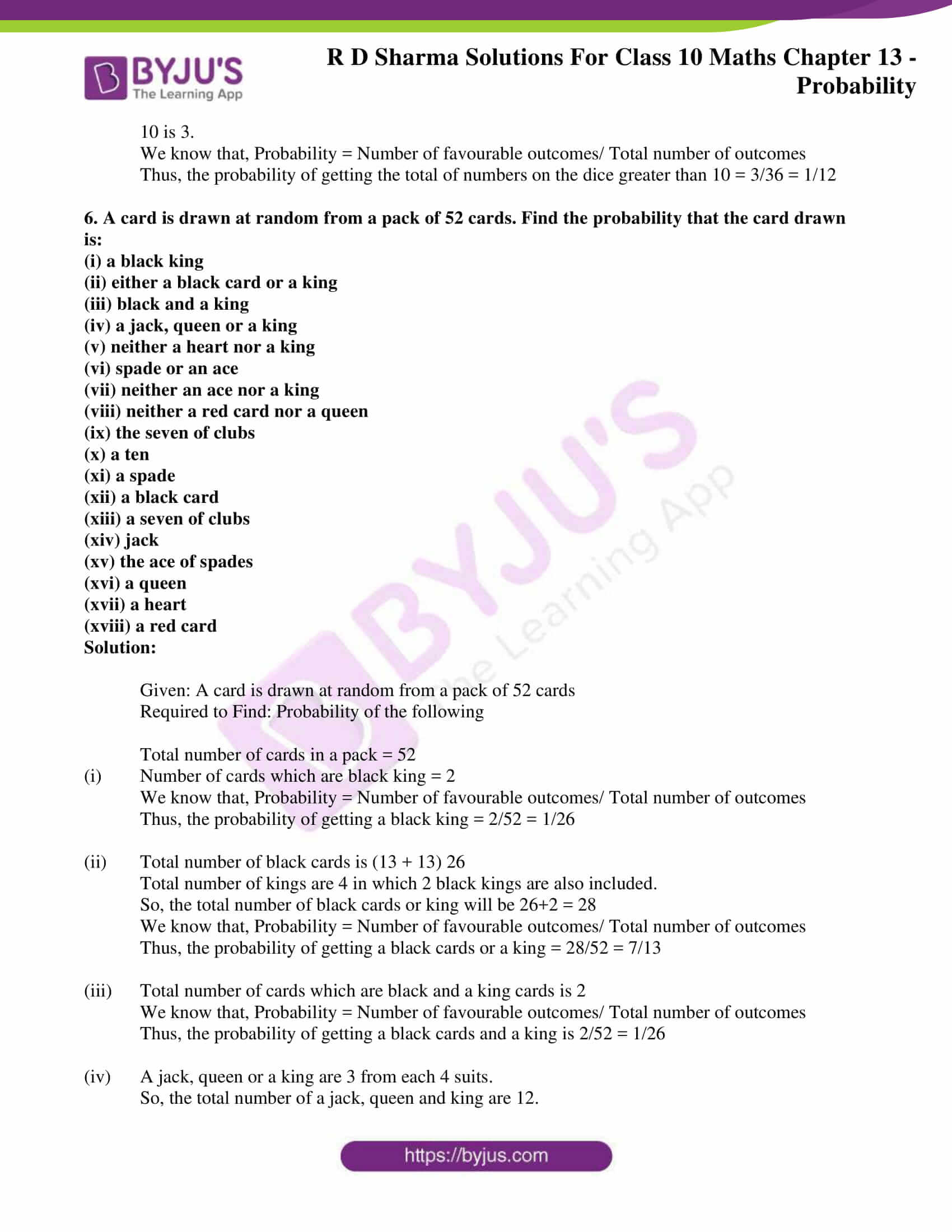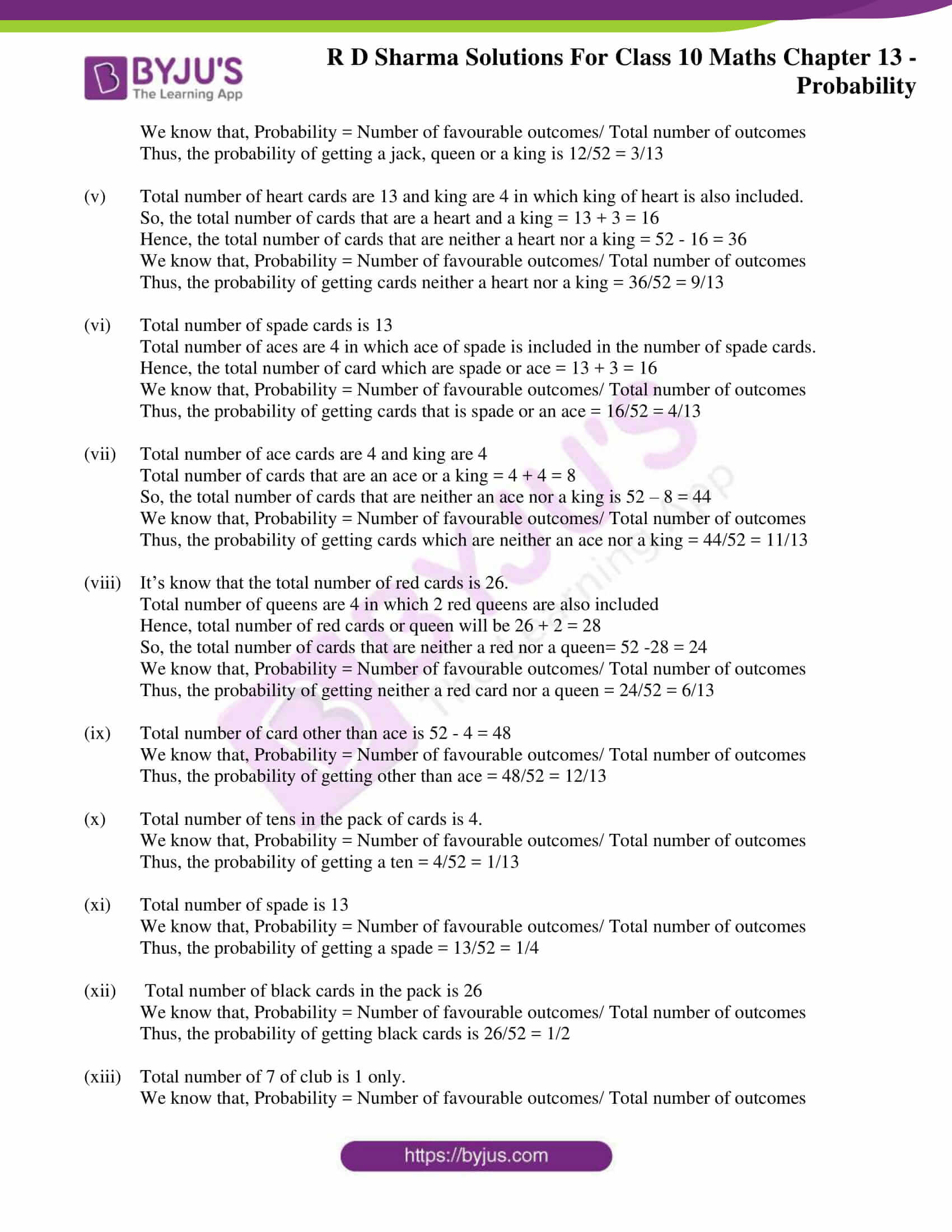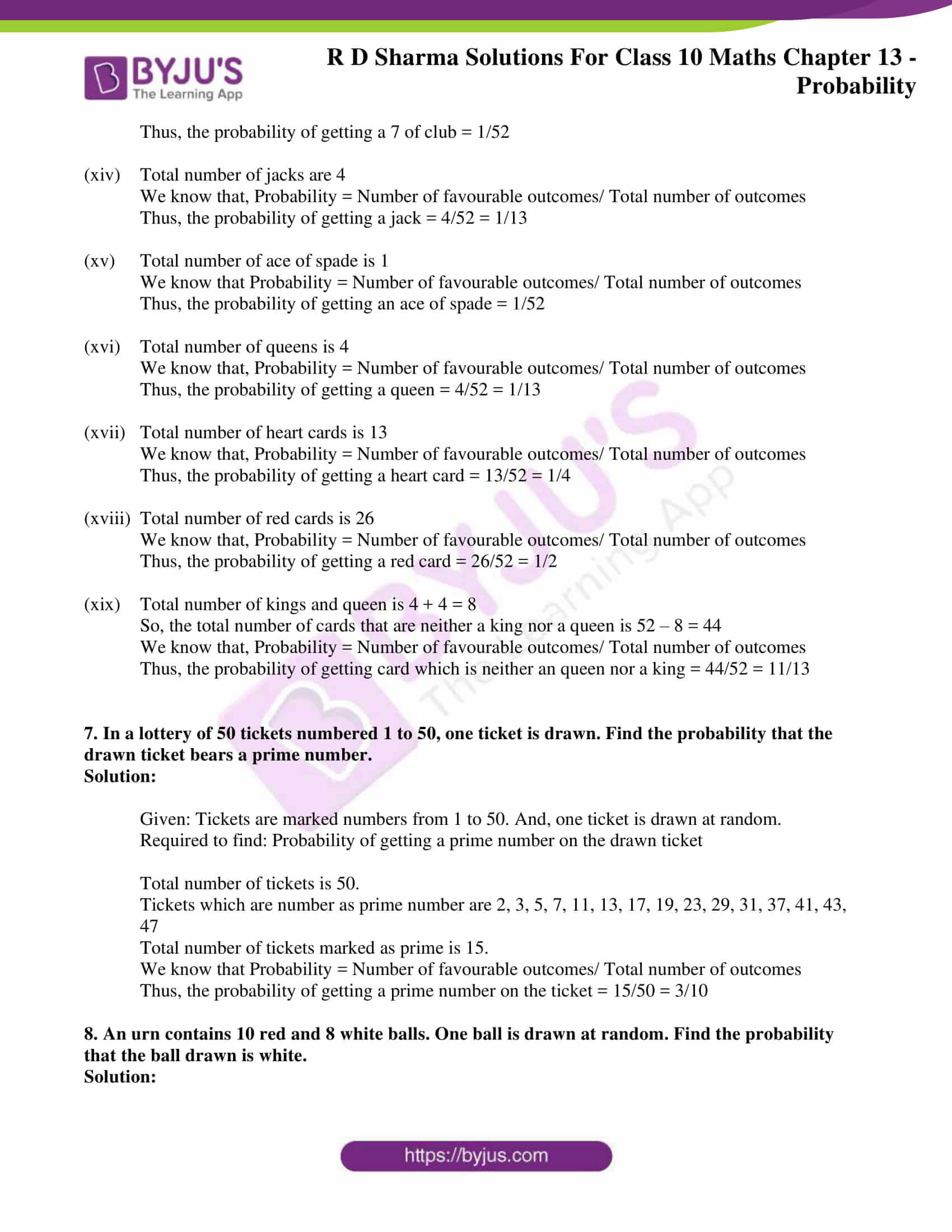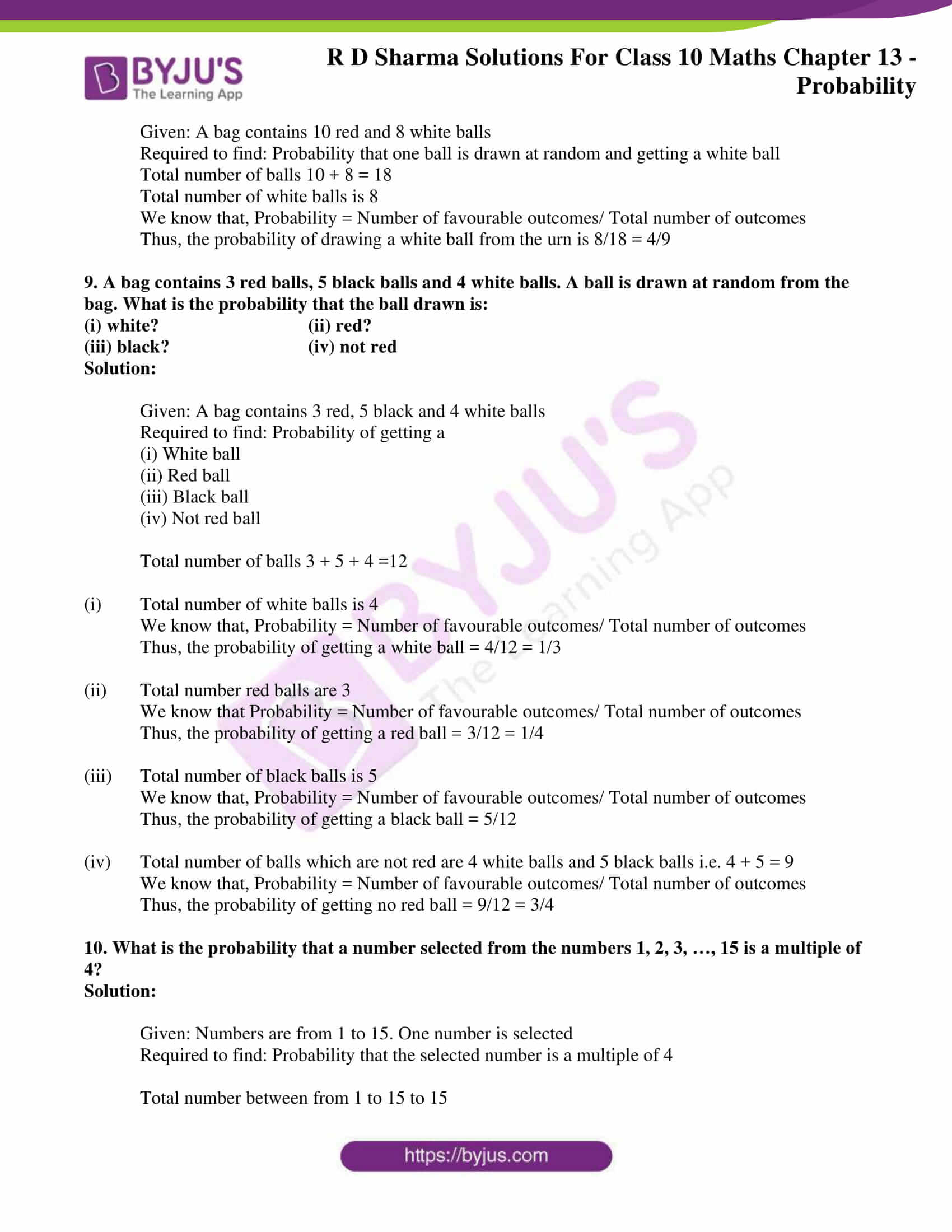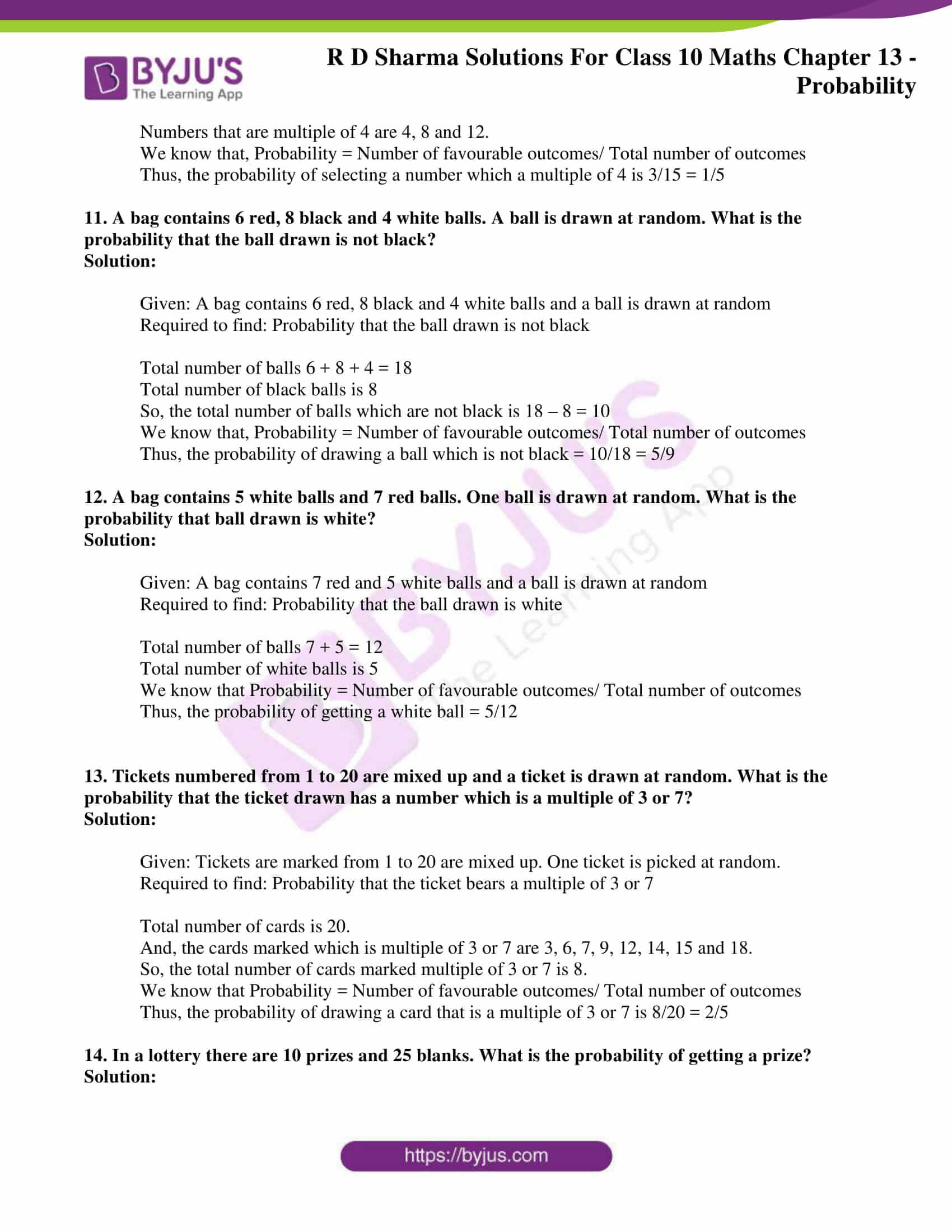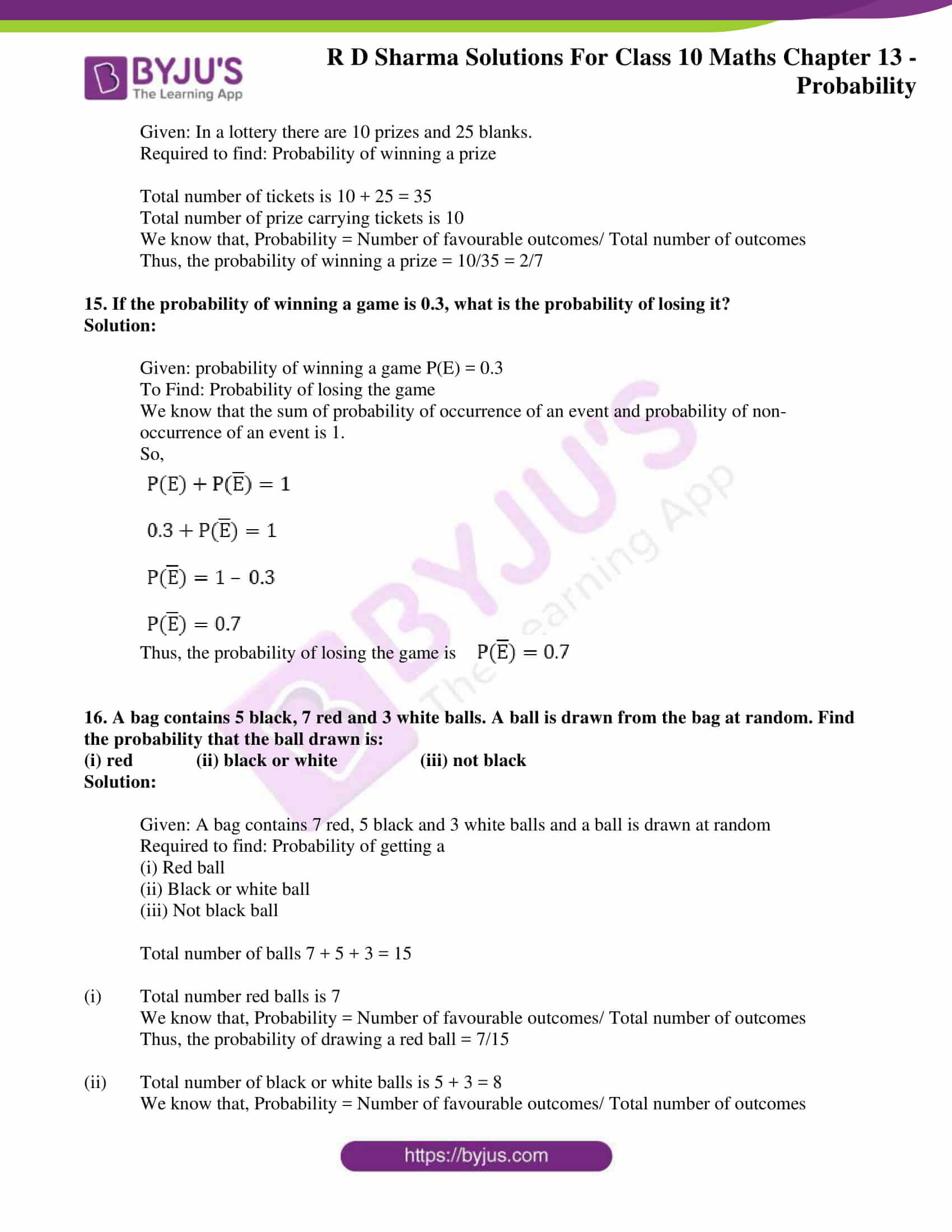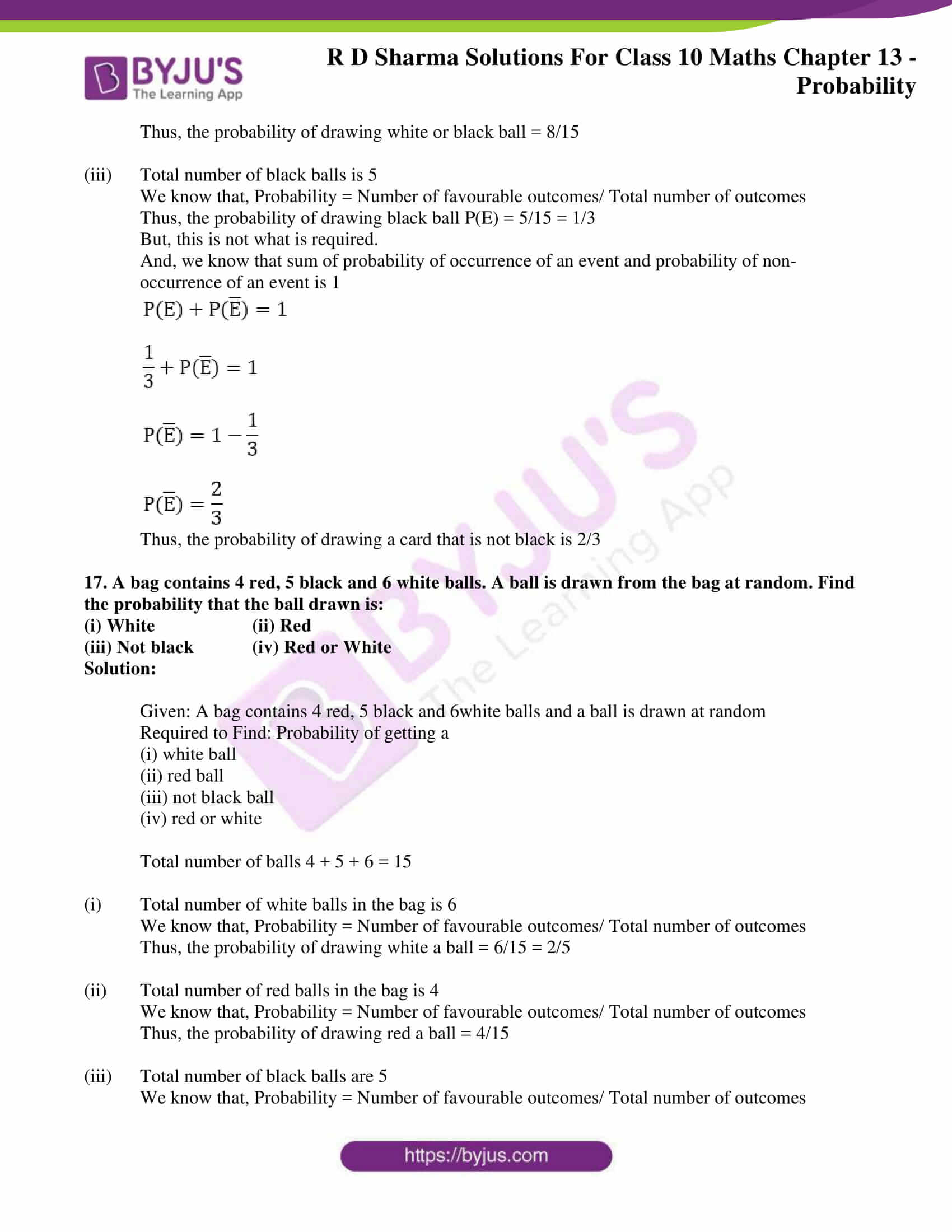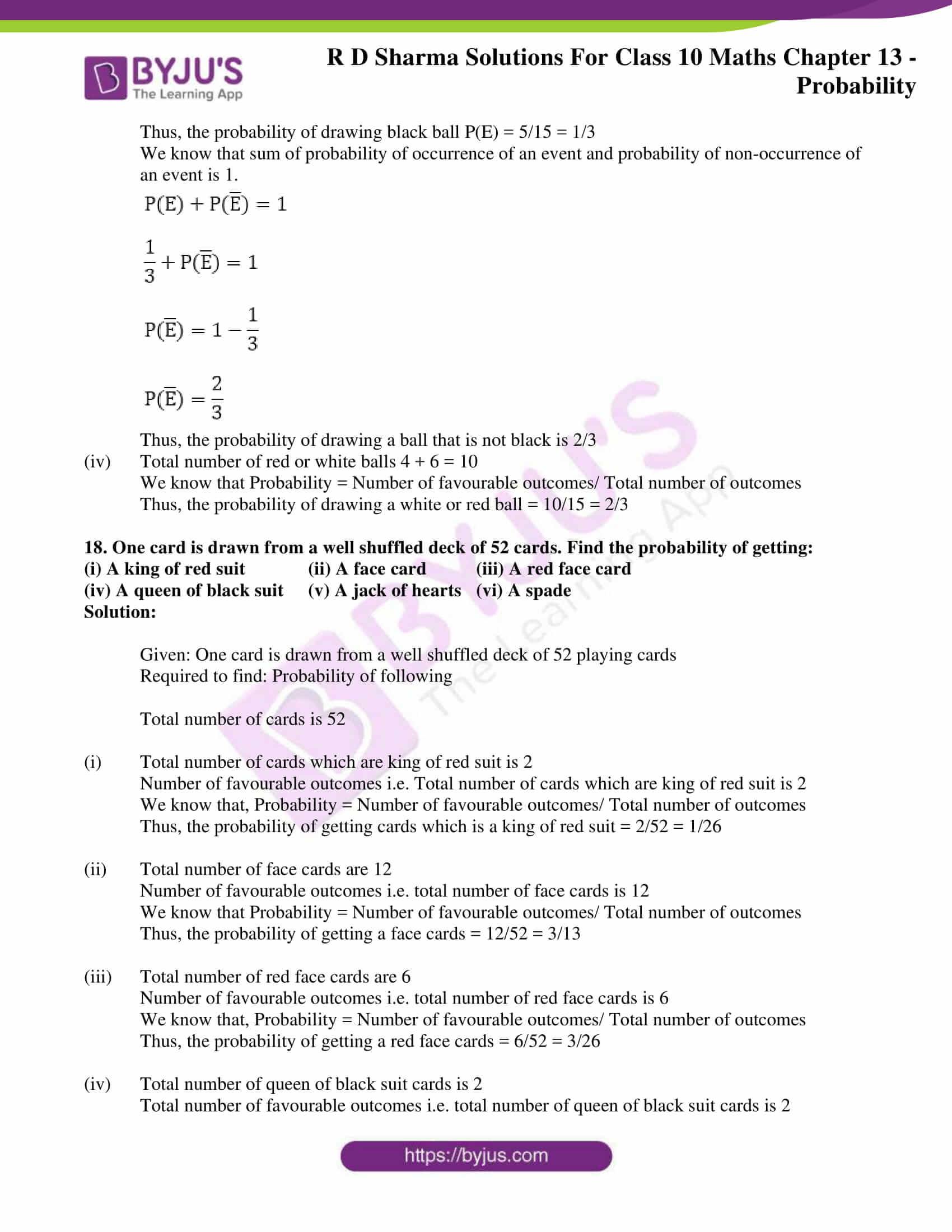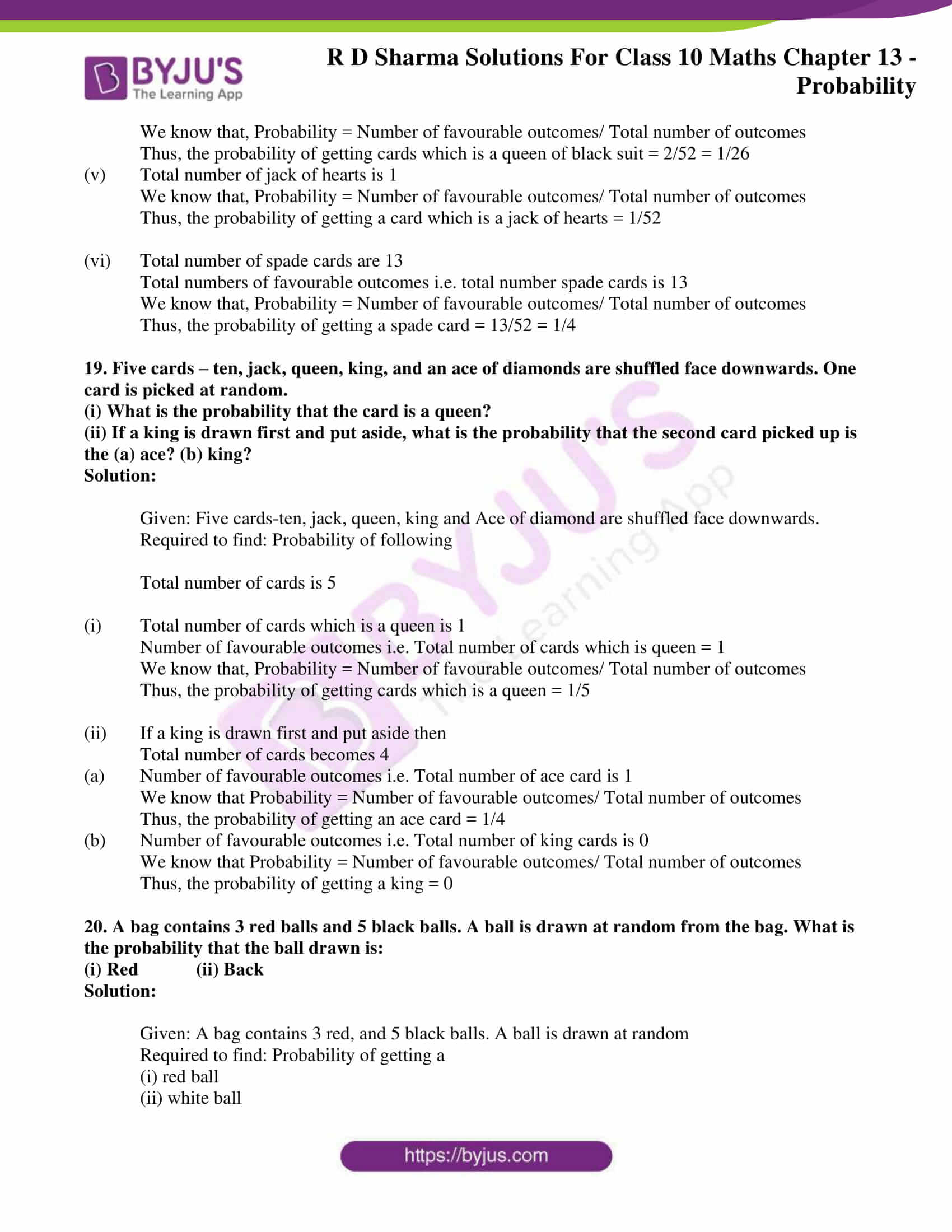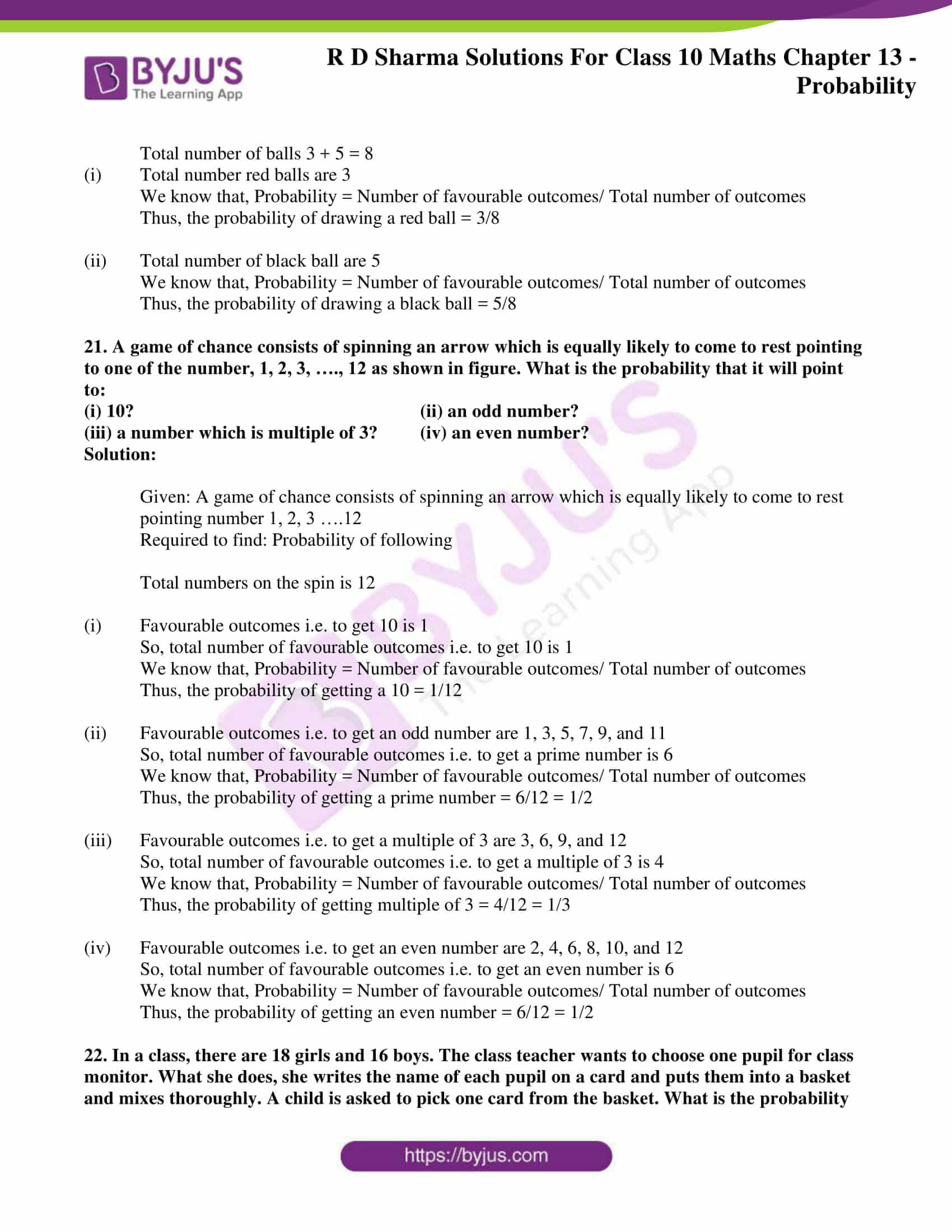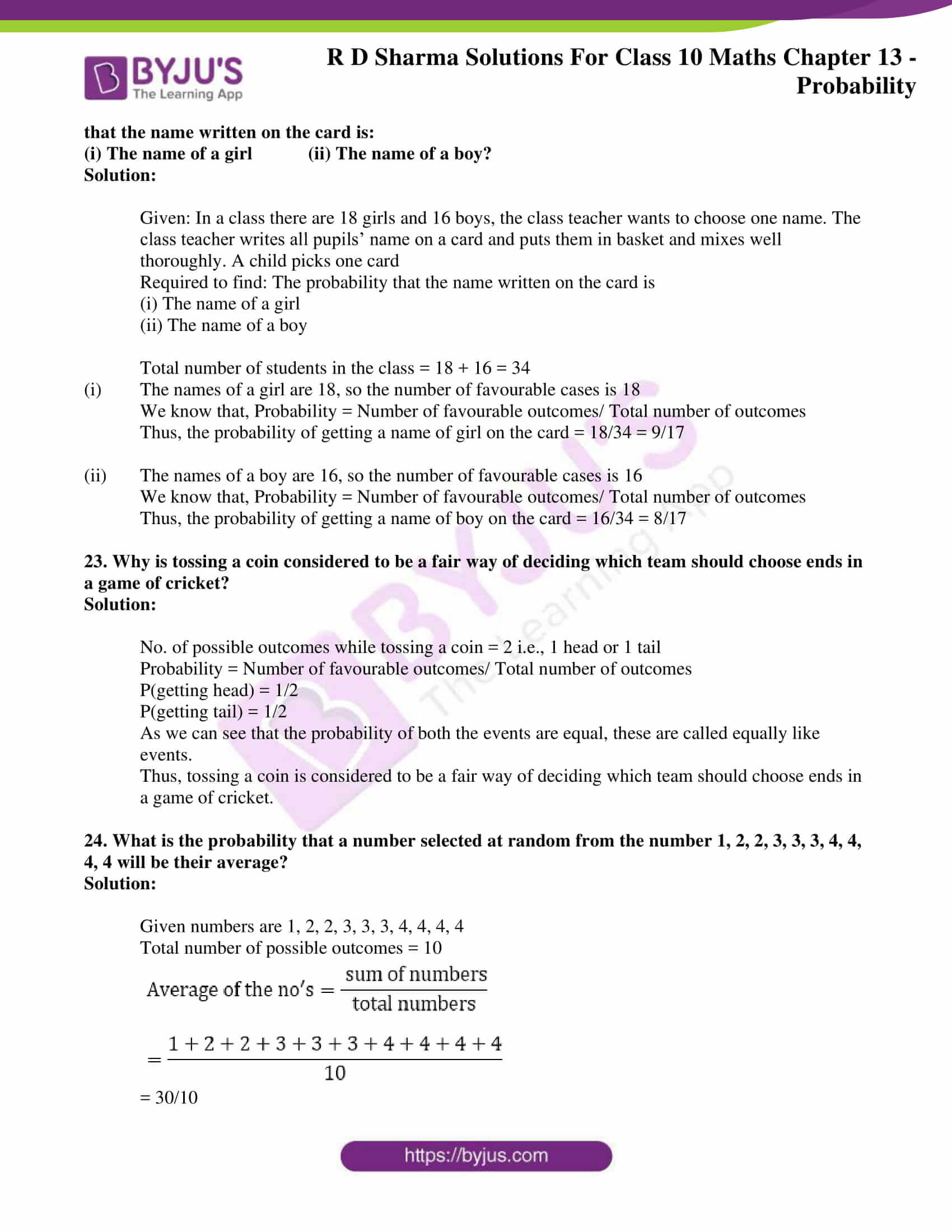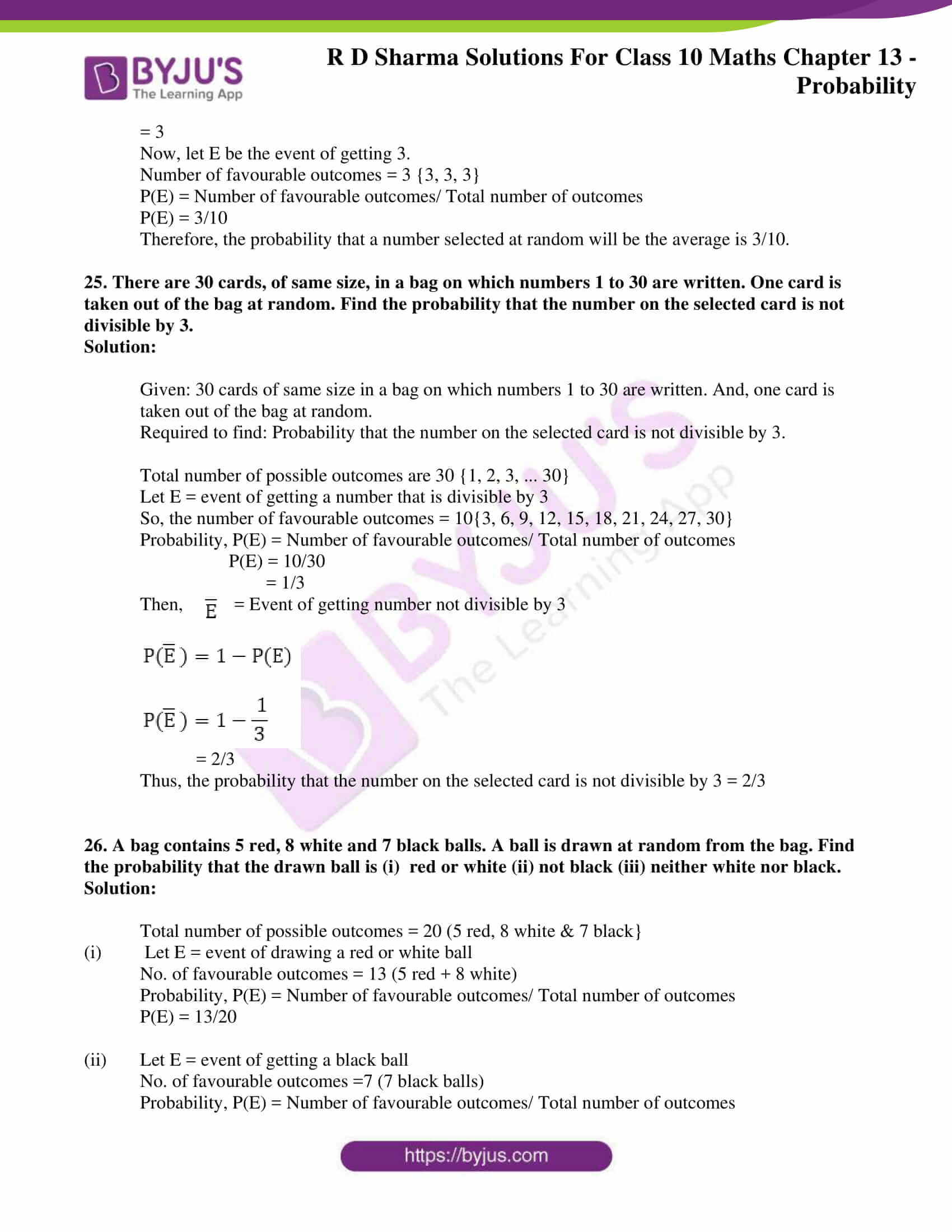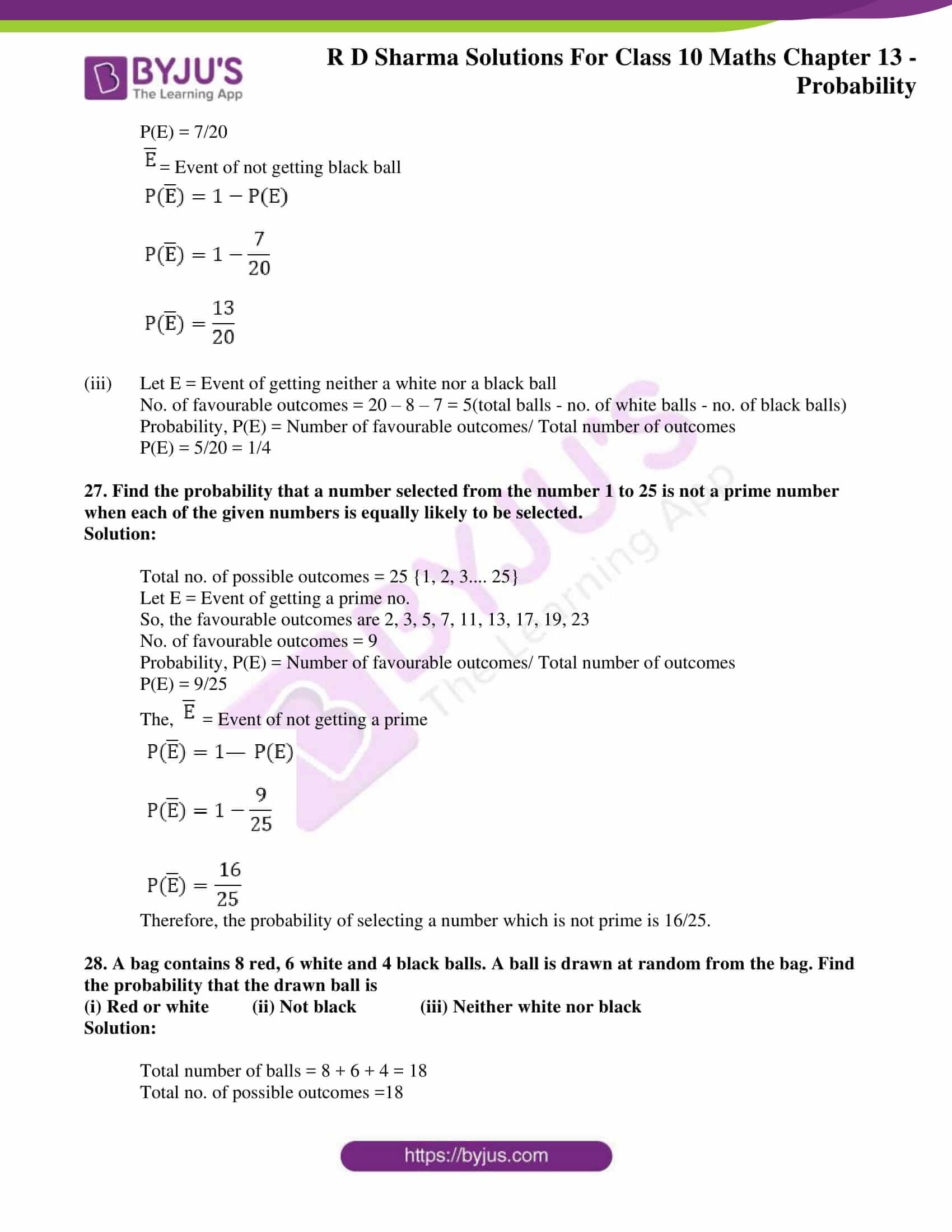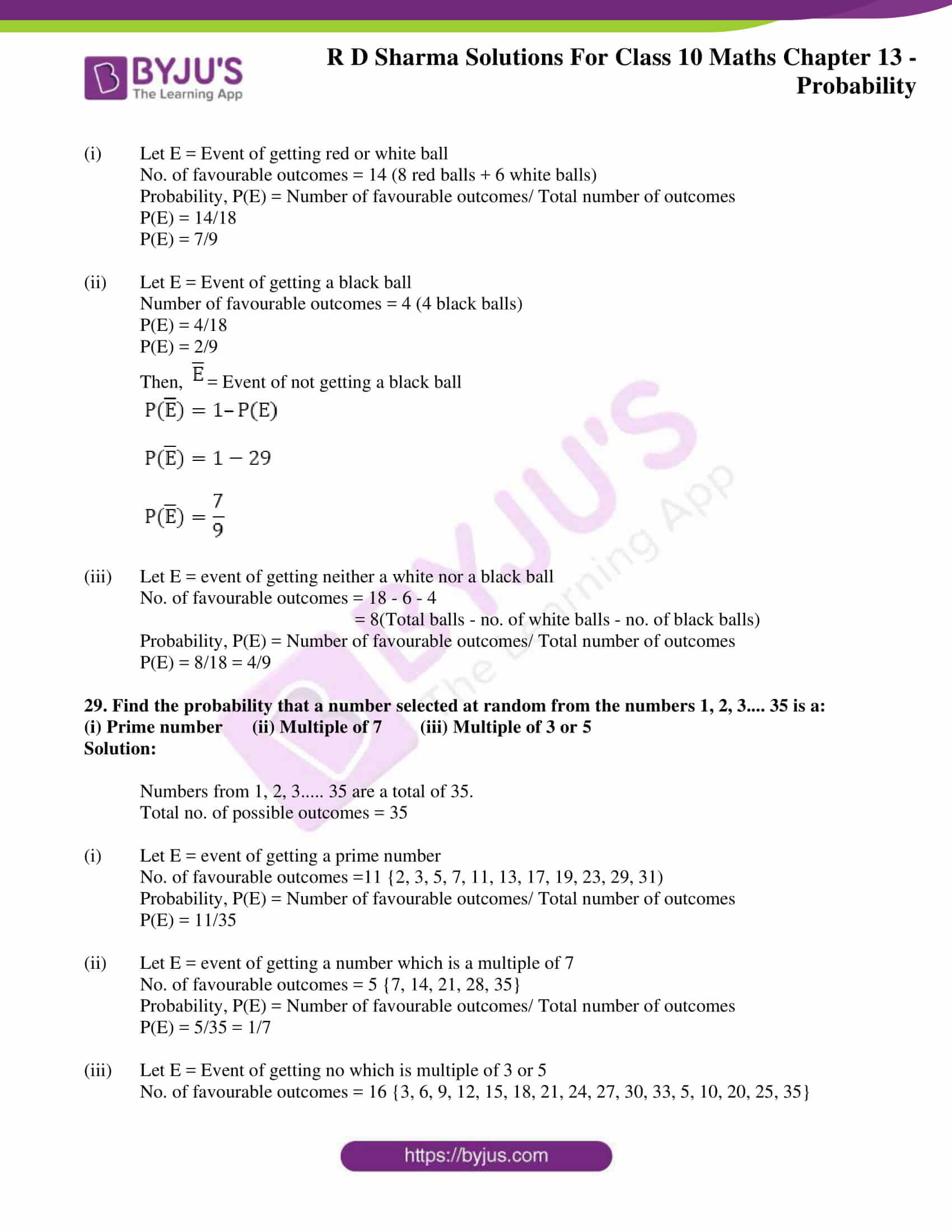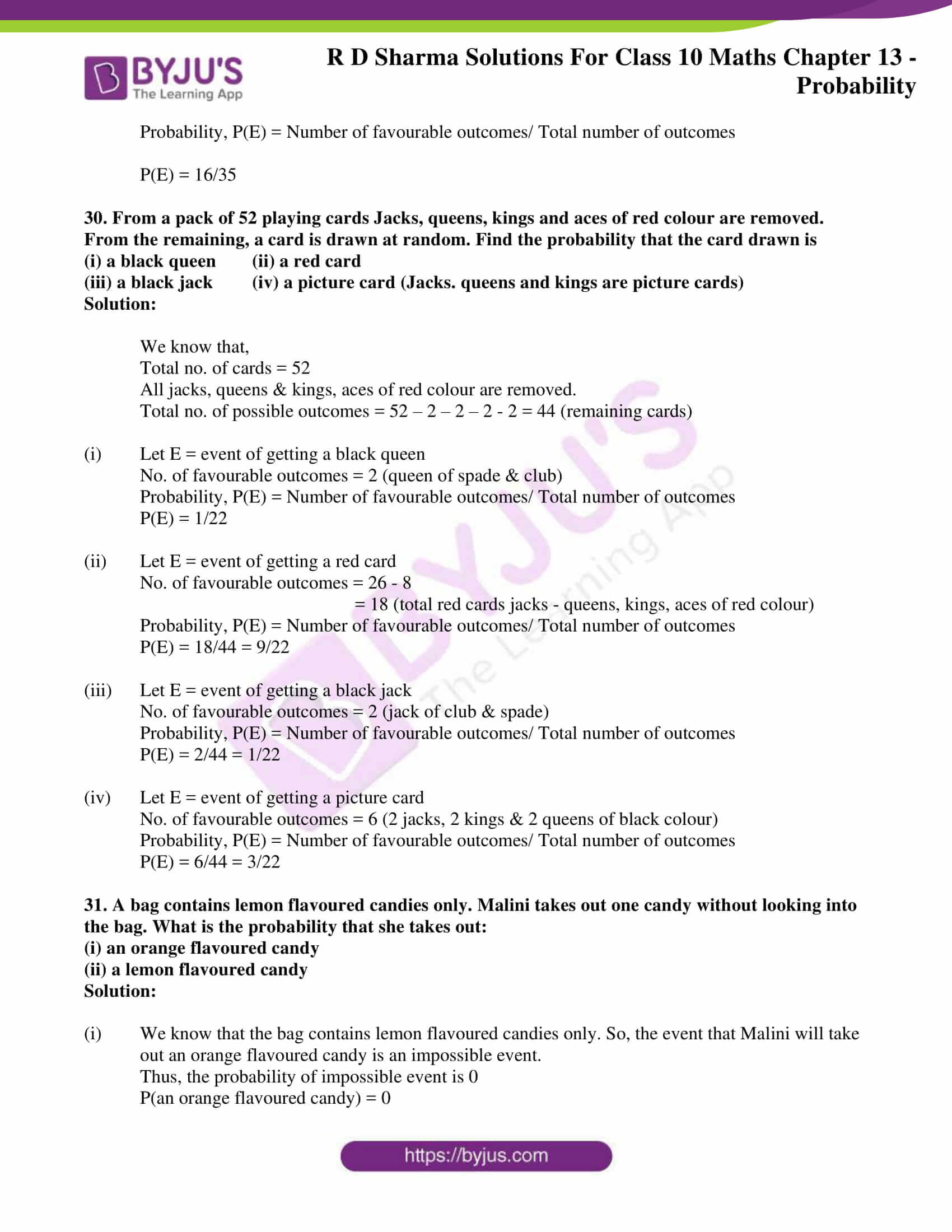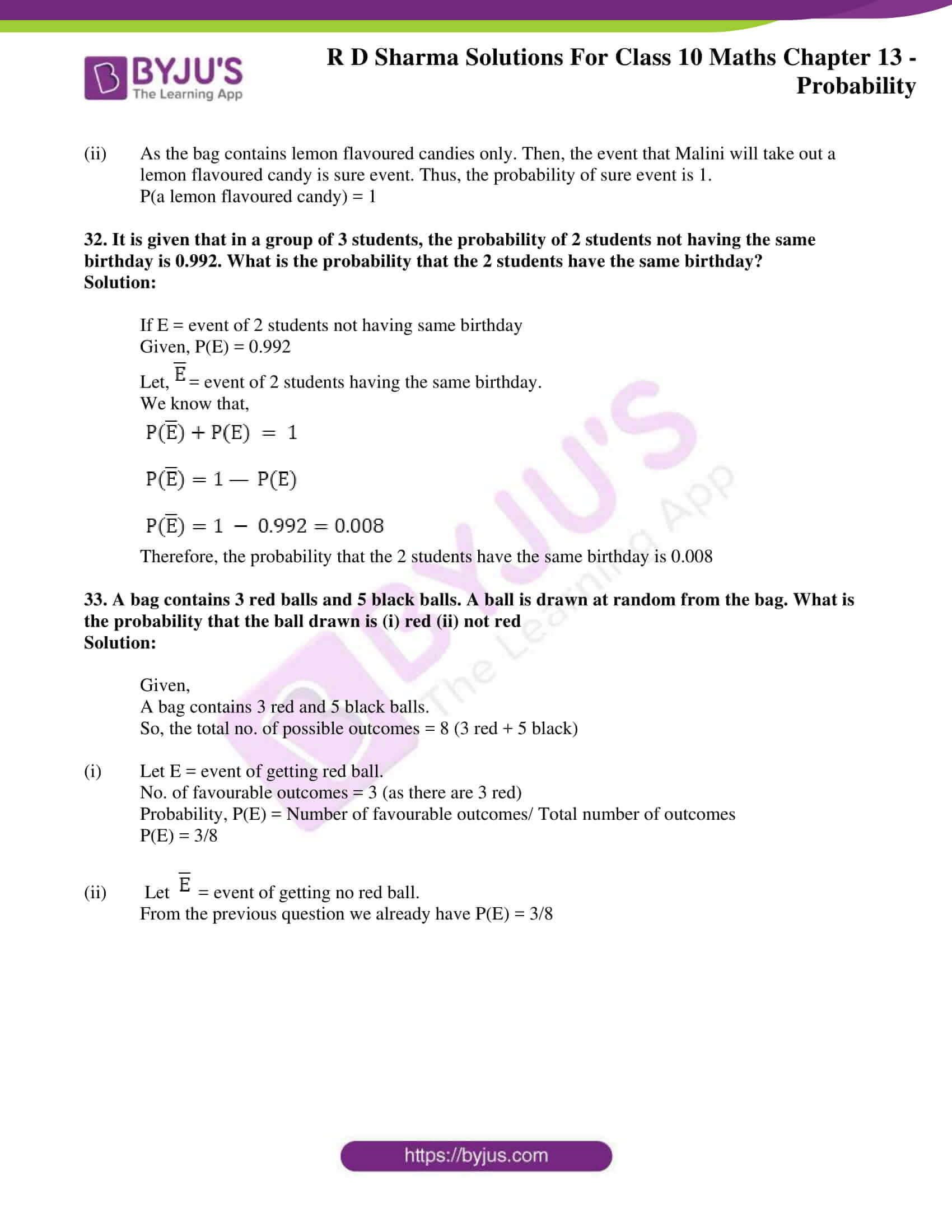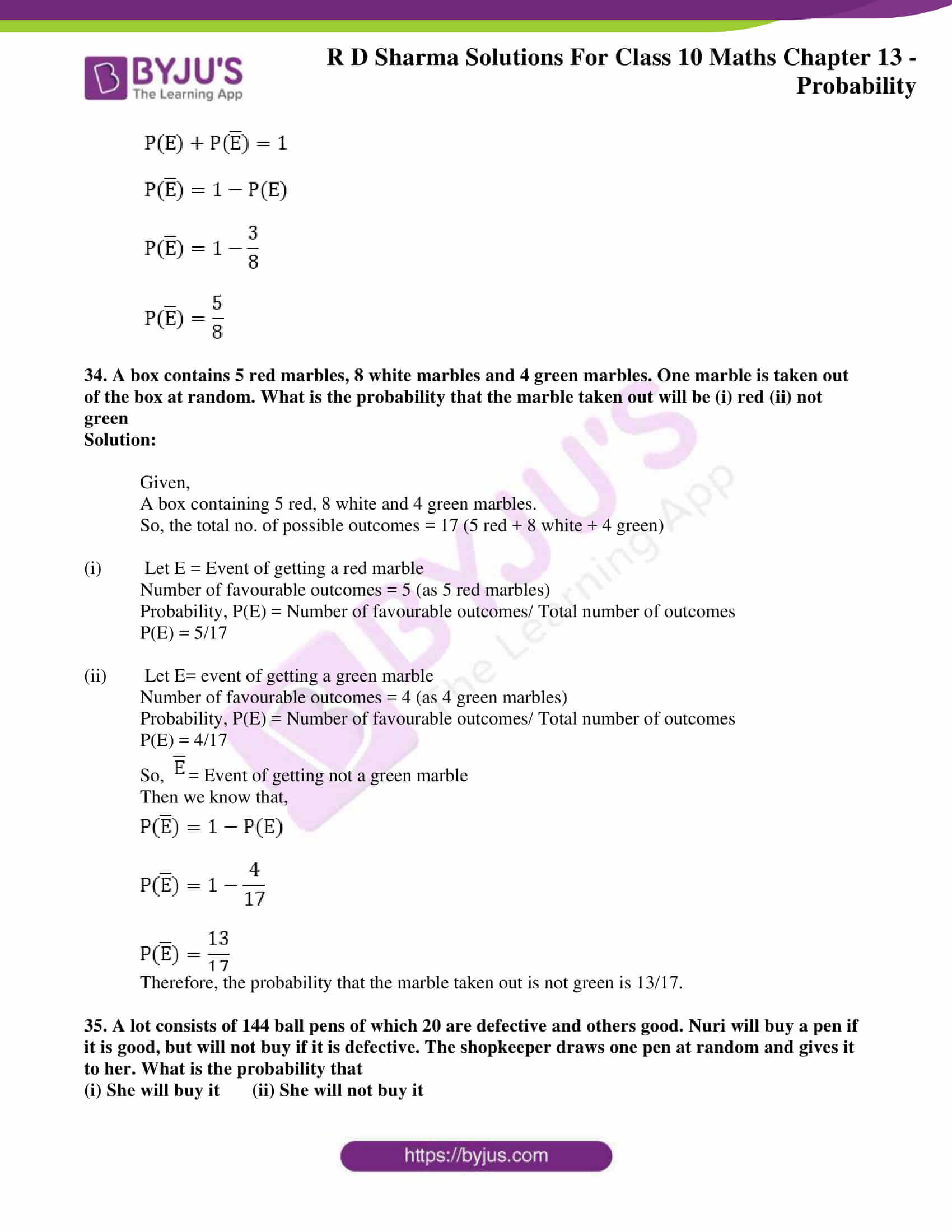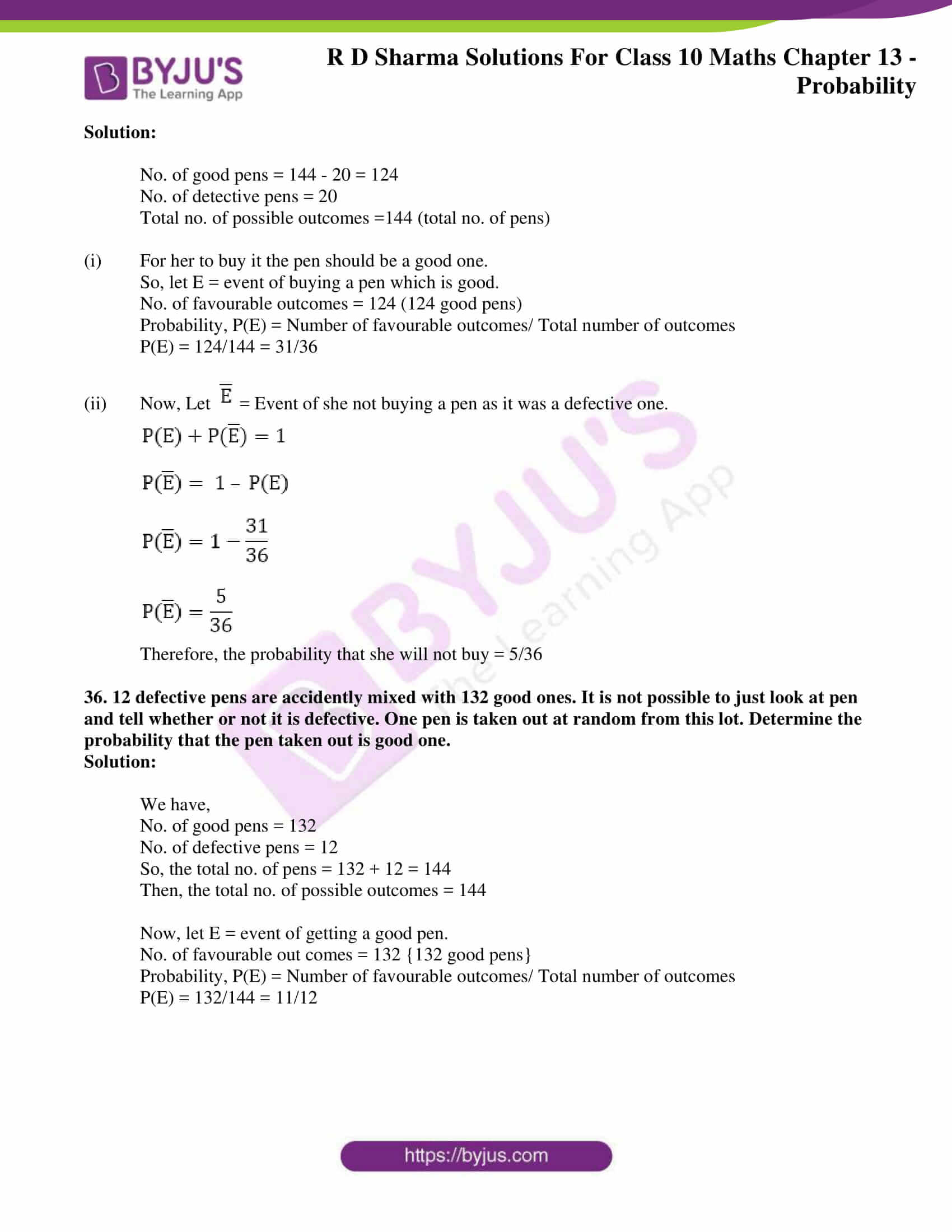### Access RD Sharma Solutions for Class 10 Maths Chapter 13 Probability Exercise 13.1

Exercise 13.1 Page No: 13.20

1. The probability that it will rain tomorrow is 0.85. What is the probability that it will not rain tomorrow?

Solution:

Given: Probability that it will rain tomorrow P(E) = 0.85

Required to find: Probability that it will not rain tomorrow P(E)

We know that sum of the probability of occurrence of an event and the probability of non-occurrence of an event is 1.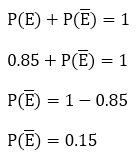Therefore, the probability that it will not rain tomorrow is = 0.15

2. A die is thrown. Find the probability of getting:

(i) a prime number   (ii) 2 or 4

(iii) a multiple of 2 or 3   (iv) an even prime number

(v) a number greater than 5           (vi) a number lying between 2 and 6

Solution:

Given: A dice is thrown once

Required to find:

(i) Probability of getting a prime number

(ii) Probability of getting 2 or 4

(iii) Probability of getting a multiple of 2 or 3.

(iv) Probability of getting an even number

(v) Probability of getting a number greater than five.

(vi) Probability of lying between 2 and 6

Total number on a dice is 6 i.e., 1, 2, 3, 4, 5 and 6.

(i) Prime numbers on a dice are 2, 3, and 5. So, the total number of prime numbers is 3.

We know that, Probability = Number of favourable outcomes/ Total number of outcomes

Thus, probability of getting a prime number = 3/6 = 1/2

(ii) For getting 2 and 4, clearly the number of favourable outcomes is 2.

We know that Probability = Number of favourable outcomes/ Total number of outcomes

Thus, the probability of getting 2 or 4 = 2/6 = 1/3

(iii) Multiple of 2 are 3 are 2, 3, 4 and 6.

So, the number of favourable outcomes is 4

We know that, Probability = Number of favourable outcomes/ Total number of outcomes

Thus, the probability of getting an multiple of 2 or 3 = 4/6 = 2/3

(iv) An even prime number is 2 only.

So, the number of favourable outcomes is 1.

We know that, Probability = Number of favourable outcomes/ Total number of outcomes

Thus, the probability of getting an even prime number = 1/6

(v) A number greater than 5 is 6 only.

So, the number of favourable outcomes is 1.

We know that, Probability = Number of favourable outcomes/ Total number of outcomes

Thus, the probability of getting a number greater than 5 = 1/6

(vi) Total number on a dice is 6.

Numbers lying between 2 and 6 are 3, 4 and 5

So, the total number of numbers lying between 2 and 6 is 3.

We know that, Probability = Number of favourable outcomes/ Total number of outcomes

Thus, the probability of getting a number lying between 2 and 6 = 3/6 =1/2

3. Three coins are tossed together. Find the probability of getting:

(iii) at least one head and one tail (iv) no tails

Solution:

Given: Three coins are tossed simultaneously.

When three coins are tossed then the outcome will be anyone of these combinations.

TTT, THT, TTH, THH. HTT, HHT, HTH, HHH.

So, the total number of outcomes is 8.

(i) For exactly two heads, the favourable outcome are THH, HHT, HTH

So, the total number of favourable outcomes is 3.

We know that, Probability = Number of favourable outcomes/ Total number of outcomes

Thus, the probability of getting exactly two heads is 3/8

(ii) For getting at least two heads the favourable outcomes are HHT, HTH, HHH, and THH

So, the total number of favourable outcomes is 4.

We know that, Probability = Number of favourable outcomes/ Total number of outcomes

Thus, the probability of getting at least two heads when three coins are tossed simultaneously = 4/8 = 1/2

(iii) For getting at least one head and one tail the cases are THT, TTH, THH, HTT, HHT, and HTH.

So, the total number of favourable outcomes i.e. at least one tail and one head is 6

We know that, Probability = Number of favourable outcomes/ Total number of outcomes

Thus, the probability of getting at least one head and one tail = 6/8 = 3/4

(iv) For getting an outcome of no tail, the only possibility is HHH.

So, the total number of favourable outcomes is 1.

We know that, Probability = Number of favourable outcomes/ Total number of outcomes

Thus, the probability of getting no tails is 1/8.

4. A and B throw a pair of dice. If A throws 9, find B’s chance of throwing a higher number

Solution:

Given A pair of dice is thrown

Required to find: Probability that the total of numbers on the dice is greater than 9

First let’s write the all possible events that can occur

(1,1), (1,2), (1,3), (1,4), (1,5), (1,6),

(2,1), (2,2), (2,3), (2,4), (2,5), (2,6),

(3,1), (3,2), (3,3), (3,4), (3,5), (3,6),

(4,1), (4,2), (4,3), (4,4), (4,5), (4,6),

(5,1), (5,2), (5,3), (5,4), (5,5), (5,6),

(6,1), (6,2), (6,3), (6,4), (6,5), (6,6),

It’s seen that the total number of events is 62 = 36

Favourable events i.e. getting the total of numbers on the dice greater than 9 are

(5,5), (5,6), (6,4), (4,6), (6,5) and (6,6).

So, the total number of favourable events i.e. getting the total of numbers on the dice greater than 9 is 6

We know that, Probability = = Number of favourable outcomes/ Total number of outcomes

Thus, the probability of getting the total of numbers on the dice greater than 9 = 6/36 = 1/6

5. Two unbiased dice are thrown. Find the probability that the total of the numbers on the dice is greater than 10.

Solution:

Given A pair of dice is thrown

Required to find: Probability that the total of numbers on the dice is greater than 10

First let’s write the all possible events that can occur

(1,1), (1,2), (1,3), (1,4), (1,5), (1,6),

(2,1), (2,2), (2,3), (2,4), (2,5), (2,6),

(3,1), (3,2), (3,3), (3,4), (3,5), (3,6),

(4,1), (4,2), (4,3), (4,4), (4,5), (4,6),

(5,1), (5,2), (5,3), (5,4), (5,5), (5,6),

(6,1), (6,2), (6,3), (6,4), (6,5), (6,6),

It’s seen that the total number of events is 62 = 36

Favourable events i.e. getting the total of numbers on the dice greater than 10 are (5, 6), (6, 5) and (6, 6).

So, the total number of favourable events i.e. getting the total of numbers on the dice greater than 10 is 3.

We know that, Probability = Number of favourable outcomes/ Total number of outcomes

Thus, the probability of getting the total of numbers on the dice greater than 10 = 3/36 = 1/12

6. A card is drawn at random from a pack of 52 cards. Find the probability that the card drawn is:

(i) a black king

(ii) either a black card or a king

(iii) black and a king

(iv) a jack, queen or a king

(v) neither a heart nor a king

(vii) neither an ace nor a king

(viii) neither a red card nor a queen

(ix) the seven of clubs

(x) a ten

(xii) a black card

(xiii) a seven of clubs

(xiv) jack

(xvi) a queen

(xvii) a heart

(xviii) a red card

Solution:

Given: A card is drawn at random from a pack of 52 cards

Required to Find: Probability of the following

Total number of cards in a pack = 52

(i) Number of cards which are black king = 2

We know that, Probability = Number of favourable outcomes/ Total number of outcomes

Thus, the probability of getting a black king = 2/52 = 1/26

(ii) Total number of black cards is (13 + 13) 26

Total number of kings are 4 in which 2 black kings are also included.

So, the total number of black cards or king will be 26+2 = 28

We know that, Probability = Number of favourable outcomes/ Total number of outcomes

Thus, the probability of getting a black cards or a king = 28/52 = 7/13

(iii) Total number of cards which are black and a king cards is 2

We know that, Probability = Number of favourable outcomes/ Total number of outcomes

Thus, the probability of getting a black cards and a king is 2/52 = 1/26

(iv) A jack, queen or a king are 3 from each 4 suits.

So, the total number of a jack, queen and king are 12.

We know that, Probability = Number of favourable outcomes/ Total number of outcomes

Thus, the probability of getting a jack, queen or a king is 12/52 = 3/13

(v) Total number of heart cards are 13 and king are 4 in which king of heart is also included.

So, the total number of cards that are a heart and a king = 13 + 3 = 16

Hence, the total number of cards that are neither a heart nor a king = 52 – 16 = 36

We know that, Probability = Number of favourable outcomes/ Total number of outcomes

Thus, the probability of getting cards neither a heart nor a king = 36/52 = 9/13

(vi) Total number of spade cards is 13

Total number of aces are 4 in which ace of spade is included in the number of spade cards.

Hence, the total number of card which are spade or ace = 13 + 3 = 16

We know that, Probability = Number of favourable outcomes/ Total number of outcomes

Thus, the probability of getting cards that is spade or an ace = 16/52 = 4/13

(vii) Total number of ace cards are 4 and king are 4

Total number of cards that are an ace or a king = 4 + 4 = 8

So, the total number of cards that are neither an ace nor a king is 52 – 8 = 44

We know that, Probability = Number of favourable outcomes/ Total number of outcomes

Thus, the probability of getting cards which are neither an ace nor a king = 44/52 = 11/13

(viii) It’s know that the total number of red cards is 26.

Total number of queens are 4 in which 2 red queens are also included

Hence, total number of red cards or queen will be 26 + 2 = 28

So, the total number of cards that are neither a red nor a queen= 52 -28 = 24

We know that, Probability = Number of favourable outcomes/ Total number of outcomes

Thus, the probability of getting neither a red card nor a queen = 24/52 = 6/13

(ix) Total number of card other than ace is 52 – 4 = 48

We know that, Probability = Number of favourable outcomes/ Total number of outcomes

Thus, the probability of getting other than ace = 48/52 = 12/13

(x) Total number of tens in the pack of cards is 4.

We know that, Probability = Number of favourable outcomes/ Total number of outcomes

Thus, the probability of getting a ten = 4/52 = 1/13

(xi) Total number of spade is 13

We know that, Probability = Number of favourable outcomes/ Total number of outcomes

Thus, the probability of getting a spade = 13/52 = 1/4

(xii) Total number of black cards in the pack is 26

We know that, Probability = Number of favourable outcomes/ Total number of outcomes

Thus, the probability of getting black cards is 26/52 = 1/2

(xiii) Total number of 7 of club is 1 only.

We know that, Probability = Number of favourable outcomes/ Total number of outcomes

Thus, the probability of getting a 7 of club = 1/52

(xiv) Total number of jacks are 4

We know that, Probability = Number of favourable outcomes/ Total number of outcomes

Thus, the probability of getting a jack = 4/52 = 1/13

(xv) Total number of ace of spade is 1

We know that Probability = Number of favourable outcomes/ Total number of outcomes

Thus, the probability of getting an ace of spade = 1/52

(xvi) Total number of queens is 4

We know that, Probability = Number of favourable outcomes/ Total number of outcomes

Thus, the probability of getting a queen = 4/52 = 1/13

(xvii) Total number of heart cards is 13

We know that, Probability = Number of favourable outcomes/ Total number of outcomes

Thus, the probability of getting a heart card = 13/52 = 1/4

(xviii) Total number of red cards is 26

We know that, Probability = Number of favourable outcomes/ Total number of outcomes

Thus, the probability of getting a red card = 26/52 = 1/2

(xix) Total number of kings and queen is 4 + 4 = 8

So, the total number of cards that are neither a king nor a queen is 52 – 8 = 44

We know that, Probability = Number of favourable outcomes/ Total number of outcomes

Thus, the probability of getting card which is neither an queen nor a king = 44/52 = 11/13

7. In a lottery of 50 tickets numbered 1 to 50, one ticket is drawn. Find the probability that the drawn ticket bears a prime number.

Solution:

Given: Tickets are marked numbers from 1 to 50. And, one ticket is drawn at random.

Required to find: Probability of getting a prime number on the drawn ticket

Total number of tickets is 50.

Tickets which are number as prime number are 2, 3, 5, 7, 11, 13, 17, 19, 23, 29, 31, 37, 41, 43, 47

Total number of tickets marked as prime is 15.

We know that Probability = Number of favourable outcomes/ Total number of outcomes

Thus, the probability of getting a prime number on the ticket = 15/50 = 3/10

8. An urn contains 10 red and 8 white balls. One ball is drawn at random. Find the probability that the ball drawn is white.

Solution:

Given: A bag contains 10 red and 8 white balls

Required to find: Probability that one ball is drawn at random and getting a white ball

Total number of balls 10 + 8 = 18

Total number of white balls is 8

We know that, Probability = Number of favourable outcomes/ Total number of outcomes

Thus, the probability of drawing a white ball from the urn is 8/18 = 4/9

9. A bag contains 3 red balls, 5 black balls and 4 white balls. A ball is drawn at random from the bag. What is the probability that the ball drawn is:

(i) white? (ii) red?

(iii) black? (iv) not red

Solution:

Given: A bag contains 3 red, 5 black and 4 white balls

Required to find: Probability of getting a

(i) White ball

(ii) Red ball

(iii) Black ball

(iv) Not red ball

Total number of balls 3 + 5 + 4 =12

(i) Total number of white balls is 4

We know that, Probability = Number of favourable outcomes/ Total number of outcomes

Thus, the probability of getting a white ball = 4/12 = 1/3

(ii) Total number red balls are 3

We know that Probability = Number of favourable outcomes/ Total number of outcomes

Thus, the probability of getting a red ball = 3/12 = 1/4

(iii) Total number of black balls is 5

We know that, Probability = Number of favourable outcomes/ Total number of outcomes

Thus, the probability of getting a black ball = 5/12

(iv) Total number of balls which are not red are 4 white balls and 5 black balls i.e. 4 + 5 = 9

We know that, Probability = Number of favourable outcomes/ Total number of outcomes

Thus, the probability of getting no red ball = 9/12 = 3/4

10. What is the probability that a number selected from the numbers 1, 2, 3, …, 15 is a multiple of 4?

Solution:

Given: Numbers are from 1 to 15. One number is selected

Required to find: Probability that the selected number is a multiple of 4

Total number between from 1 to 15 to 15

Numbers that are multiple of 4 are 4, 8 and 12.

We know that, Probability = Number of favourable outcomes/ Total number of outcomes

Thus, the probability of selecting a number which a multiple of 4 is 3/15 = 1/5

11. A bag contains 6 red, 8 black and 4 white balls. A ball is drawn at random. What is the probability that the ball drawn is not black?

Solution:

Given: A bag contains 6 red, 8 black and 4 white balls and a ball is drawn at random

Required to find: Probability that the ball drawn is not black

Total number of balls 6 + 8 + 4 = 18

Total number of black balls is 8

So, the total number of balls which are not black is 18 – 8 = 10

We know that, Probability = Number of favourable outcomes/ Total number of outcomes

Thus, the probability of drawing a ball which is not black = 10/18 = 5/9

12. A bag contains 5 white balls and 7 red balls. One ball is drawn at random. What is the probability that ball drawn is white?

Solution:

Given: A bag contains 7 red and 5 white balls and a ball is drawn at random

Required to find: Probability that the ball drawn is white

Total number of balls 7 + 5 = 12

Total number of white balls is 5

We know that Probability = Number of favourable outcomes/ Total number of outcomes

Thus, the probability of getting a white ball = 5/12

13. Tickets numbered from 1 to 20 are mixed up and a ticket is drawn at random. What is the probability that the ticket drawn has a number which is a multiple of 3 or 7?

Solution:

Given: Tickets are marked from 1 to 20 are mixed up. One ticket is picked at random.

Required to find: Probability that the ticket bears a multiple of 3 or 7

Total number of cards is 20.

And, the cards marked which is multiple of 3 or 7 are 3, 6, 7, 9, 12, 14, 15 and 18.

So, the total number of cards marked multiple of 3 or 7 is 8.

We know that Probability = Number of favourable outcomes/ Total number of outcomes

Thus, the probability of drawing a card that is a multiple of 3 or 7 is 8/20 = 2/5

14. In a lottery there are 10 prizes and 25 blanks. What is the probability of getting a prize?

Solution:

Given: In a lottery there are 10 prizes and 25 blanks.

Required to find: Probability of winning a prize

Total number of tickets is 10 + 25 = 35

Total number of prize carrying tickets is 10

We know that, Probability = Number of favourable outcomes/ Total number of outcomes

Thus, the probability of winning a prize = 10/35 = 2/7

15. If the probability of winning a game is 0.3, what is the probability of losing it?

Solution:

Given: probability of winning a game P(E) = 0.3

To Find: Probability of losing the game

We know that the sum of probability of occurrence of an event and probability of non-occurrence of an event is 1.

So,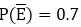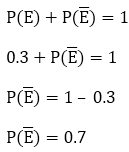Thus, the probability of losing the game is

16. A bag contains 5 black, 7 red and 3 white balls. A ball is drawn from the bag at random. Find the probability that the ball drawn is:

(i) red (ii) black or white (iii) not black

Solution:

Given: A bag contains 7 red, 5 black and 3 white balls and a ball is drawn at random

Required to find: Probability of getting a

(i) Red ball

(ii) Black or white ball

(iii) Not black ball

Total number of balls 7 + 5 + 3 = 15

(i) Total number red balls is 7

We know that, Probability = Number of favourable outcomes/ Total number of outcomes

Thus, the probability of drawing a red ball = 7/15

(ii) Total number of black or white balls is 5 + 3 = 8

We know that, Probability = Number of favourable outcomes/ Total number of outcomes

Thus, the probability of drawing white or black ball = 8/15

(iii) Total number of black balls is 5

We know that, Probability = Number of favourable outcomes/ Total number of outcomes

Thus, the probability of drawing black ball P(E) = 5/15 = 1/3

But, this is not what is required.

And, we know that sum of probability of occurrence of an event and probability of non-occurrence of an event is 1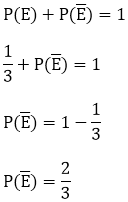Thus, the probability of drawing a card that is not black is 2/3

17. A bag contains 4 red, 5 black and 6 white balls. A ball is drawn from the bag at random. Find the probability that the ball drawn is:

(i) White (ii) Red

(iii) Not black (iv) Red or White

Solution:

Given: A bag contains 4 red, 5 black and 6white balls and a ball is drawn at random

Required to Find: Probability of getting a

(i) white ball

(ii) red ball

(iii) not black ball

(iv) red or white

Total number of balls 4 + 5 + 6 = 15

(i) Total number of white balls in the bag is 6

We know that, Probability = Number of favourable outcomes/ Total number of outcomes

Thus, the probability of drawing white a ball = 6/15 = 2/5

(ii) Total number of red balls in the bag is 4

We know that, Probability = Number of favourable outcomes/ Total number of outcomes

Thus, the probability of drawing red a ball = 4/15

(iii) Total number of black balls are 5

We know that, Probability = Number of favourable outcomes/ Total number of outcomes

Thus, the probability of drawing black ball P(E) = 5/15 = 1/3

We know that sum of probability of occurrence of an event and probability of non-occurrence of an event is 1.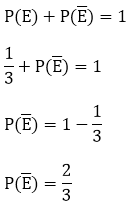Thus, the probability of drawing a ball that is not black is 2/3

(iv) Total number of red or white balls 4 + 6 = 10

We know that Probability = Number of favourable outcomes/ Total number of outcomes

Thus, the probability of drawing a white or red ball = 10/15 = 2/3

18. One card is drawn from a well shuffled deck of 52 cards. Find the probability of getting:

(i) A king of red suit (ii) A face card (iii) A red face card

(iv) A queen of black suit (v) A jack of hearts (vi) A spade

Solution:

Given: One card is drawn from a well shuffled deck of 52 playing cards

Required to find: Probability of following

Total number of cards is 52

(i) Total number of cards which are king of red suit is 2

Number of favourable outcomes i.e. Total number of cards which are king of red suit is 2

We know that, Probability = Number of favourable outcomes/ Total number of outcomes

Thus, the probability of getting cards which is a king of red suit = 2/52 = 1/26

(ii) Total number of face cards are 12

Number of favourable outcomes i.e. total number of face cards is 12

We know that Probability = Number of favourable outcomes/ Total number of outcomes

Thus, the probability of getting a face cards = 12/52 = 3/13

(iii) Total number of red face cards are 6

Number of favourable outcomes i.e. total number of red face cards is 6

We know that, Probability = Number of favourable outcomes/ Total number of outcomes

Thus, the probability of getting a red face cards = 6/52 = 3/26

(iv) Total number of queen of black suit cards is 2

Total number of favourable outcomes i.e. total number of queen of black suit cards is 2

We know that, Probability = Number of favourable outcomes/ Total number of outcomes

Thus, the probability of getting cards which is a queen of black suit = 2/52 = 1/26

(v) Total number of jack of hearts is 1

We know that, Probability = Number of favourable outcomes/ Total number of outcomes

Thus, the probability of getting a card which is a jack of hearts = 1/52

(vi) Total number of spade cards are 13

Total numbers of favourable outcomes i.e. total number spade cards is 13

We know that, Probability = Number of favourable outcomes/ Total number of outcomes

Thus, the probability of getting a spade card = 13/52 = 1/4

19. Five cards – ten, jack, queen, king, and an ace of diamonds are shuffled face downwards. One card is picked at random.

(i) What is the probability that the card is a queen?

(ii) If a king is drawn first and put aside, what is the probability that the second card picked up is the (a) ace? (b) king?

Solution:

Given: Five cards-ten, jack, queen, king and Ace of diamond are shuffled face downwards.

Required to find: Probability of following

Total number of cards is 5

(i) Total number of cards which is a queen is 1

Number of favourable outcomes i.e. Total number of cards which is queen = 1

We know that, Probability = Number of favourable outcomes/ Total number of outcomes

Thus, the probability of getting cards which is a queen = 1/5

(ii) If a king is drawn first and put aside then

Total number of cards becomes 4

(a) Number of favourable outcomes i.e. Total number of ace card is 1

We know that Probability = Number of favourable outcomes/ Total number of outcomes

Thus, the probability of getting an ace card = 1/4

(b) Number of favourable outcomes i.e. Total number of king cards is 0

We know that Probability = Number of favourable outcomes/ Total number of outcomes

Thus, the probability of getting a king = 0

20. A bag contains 3 red balls and 5 black balls. A ball is drawn at random from the bag. What is the probability that the ball drawn is:

(i) Red (ii) Back

Solution:

Given: A bag contains 3 red, and 5 black balls. A ball is drawn at random

Required to find: Probability of getting a

(i) red ball

(ii) white ball

Total number of balls 3 + 5 = 8

(i) Total number red balls are 3

We know that, Probability = Number of favourable outcomes/ Total number of outcomes

Thus, the probability of drawing a red ball = 3/8

(ii) Total number of black ball are 5

We know that, Probability = Number of favourable outcomes/ Total number of outcomes

Thus, the probability of drawing a black ball = 5/8

21. A game of chance consists of spinning an arrow which is equally likely to come to rest pointing to one of the number, 1, 2, 3, …., 12 as shown in figure. What is the probability that it will point to:

(i) 10? (ii) an odd number?

(iii) a number which is multiple of 3? (iv) an even number?

Solution:

Given: A game of chance consists of spinning an arrow which is equally likely to come to rest pointing number 1, 2, 3 ….12

Required to find: Probability of following

Total numbers on the spin is 12

(i) Favourable outcomes i.e. to get 10 is 1

So, total number of favourable outcomes i.e. to get 10 is 1

We know that, Probability = Number of favourable outcomes/ Total number of outcomes

Thus, the probability of getting a 10 = 1/12

(ii) Favourable outcomes i.e. to get an odd number are 1, 3, 5, 7, 9, and 11

So, total number of favourable outcomes i.e. to get a prime number is 6

We know that, Probability = Number of favourable outcomes/ Total number of outcomes

Thus, the probability of getting a prime number = 6/12 = 1/2

(iii) Favourable outcomes i.e. to get a multiple of 3 are 3, 6, 9, and 12

So, total number of favourable outcomes i.e. to get a multiple of 3 is 4

We know that, Probability = Number of favourable outcomes/ Total number of outcomes

Thus, the probability of getting multiple of 3 = 4/12 = 1/3

(iv) Favourable outcomes i.e. to get an even number are 2, 4, 6, 8, 10, and 12

So, total number of favourable outcomes i.e. to get an even number is 6

We know that, Probability = Number of favourable outcomes/ Total number of outcomes

Thus, the probability of getting an even number = 6/12 = 1/2

22. In a class, there are 18 girls and 16 boys. The class teacher wants to choose one pupil for class monitor. What she does, she writes the name of each pupil on a card and puts them into a basket and mixes thoroughly. A child is asked to pick one card from the basket. What is the probability that the name written on the card is:

(i) The name of a girl (ii) The name of a boy?

Solution:

Given: In a class there are 18 girls and 16 boys, the class teacher wants to choose one name. The class teacher writes all pupils’ name on a card and puts them in basket and mixes well thoroughly. A child picks one card

Required to find: The probability that the name written on the card is

(i) The name of a girl

(ii) The name of a boy

Total number of students in the class = 18 + 16 = 34

(i) The names of a girl are 18, so the number of favourable cases is 18

We know that, Probability = Number of favourable outcomes/ Total number of outcomes

Thus, the probability of getting a name of girl on the card = 18/34 = 9/17

(ii) The names of a boy are 16, so the number of favourable cases is 16

We know that, Probability = Number of favourable outcomes/ Total number of outcomes

Thus, the probability of getting a name of boy on the card = 16/34 = 8/17

23. Why is tossing a coin considered to be a fair way of deciding which team should choose ends in a game of cricket?

Solution:

No. of possible outcomes while tossing a coin = 2 i.e., 1 head or 1 tail

Probability = Number of favourable outcomes/ Total number of outcomes

P(getting tail) = 1/2

As we can see that the probability of both the events are equal, these are called equally like events.

Thus, tossing a coin is considered to be a fair way of deciding which team should choose ends in a game of cricket.

24. What is the probability that a number selected at random from the number 1, 2, 2, 3, 3, 3, 4, 4, 4, 4 will be their average?

Solution:

Given numbers are 1, 2, 2, 3, 3, 3, 4, 4, 4, 4

Total number of possible outcomes = 10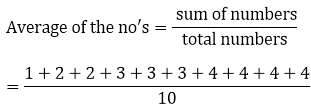= 30/10

= 3

Now, let E be the event of getting 3.

Number of favourable outcomes = 3 {3, 3, 3}

P(E) = Number of favourable outcomes/ Total number of outcomes

P(E) = 3/10

Therefore, the probability that a number selected at random will be the average is 3/10.

25. There are 30 cards, of same size, in a bag on which numbers 1 to 30 are written. One card is taken out of the bag at random. Find the probability that the number on the selected card is not divisible by 3.

Solution:

Given: 30 cards of same size in a bag on which numbers 1 to 30 are written. And, one card is taken out of the bag at random.

Required to find: Probability that the number on the selected card is not divisible by 3.

Total number of possible outcomes are 30 {1, 2, 3, … 30}

Let E = event of getting a number that is divisible by 3

So, the number of favourable outcomes = 10{3, 6, 9, 12, 15, 18, 21, 24, 27, 30}

Probability, P(E) = Number of favourable outcomes/ Total number of outcomes

P(E) = 10/30

= 1/3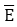Then, = Event of getting number not divisible by 3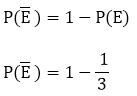= 2/3

Thus, the probability that the number on the selected card is not divisible by 3 = 2/3

26. A bag contains 5 red, 8 white and 7 black balls. A ball is drawn at random from the bag. Find the probability that the drawn ball is (i)  red or white (ii) not black (iii) neither white nor black.

Solution:

Total number of possible outcomes = 20 (5 red, 8 white & 7 black}

(i)  Let E = event of drawing a red or white ball

No. of favourable outcomes = 13 (5 red + 8 white)

Probability, P(E) = Number of favourable outcomes/ Total number of outcomes

P(E) = 13/20

(ii) Let E = event of getting a black ball

No. of favourable outcomes =7 (7 black balls)

Probability, P(E) = Number of favourable outcomes/ Total number of outcomes

P(E) = 7/20= Event of not getting black ball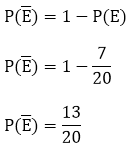(iii) Let E = Event of getting neither a white nor a black ball

No. of favourable outcomes = 20 – 8 – 7 = 5(total balls – no. of white balls – no. of black balls)

Probability, P(E) = Number of favourable outcomes/ Total number of outcomes

P(E) = 5/20 = 1/4

27. Find the probability that a number selected from the number 1 to 25 is not a prime number when each of the given numbers is equally likely to be selected.

Solution:

Total no. of possible outcomes = 25 {1, 2, 3…. 25}

Let E = Event of getting a prime no.

So, the favourable outcomes are 2, 3, 5, 7, 11, 13, 17, 19, 23

No. of favourable outcomes = 9

Probability, P(E) = Number of favourable outcomes/ Total number of outcomes

P(E) = 9/25

The,= Event of not getting a prime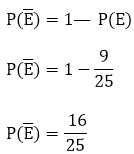Therefore, the probability of selecting a number which is not prime is 16/25.

28. A bag contains 8 red, 6 white and 4 black balls. A ball is drawn at random from the bag. Find the probability that the drawn ball is

(i) Red or white (ii) Not black (iii) Neither white nor black

Solution:

Total number of balls = 8 + 6 + 4 = 18

Total no. of possible outcomes =18

(i)  Let E = Event of getting red or white ball

No. of favourable outcomes = 14 (8 red balls + 6 white balls)

Probability, P(E) = Number of favourable outcomes/ Total number of outcomes

P(E) = 14/18

P(E) = 7/9

(ii)  Let E = Event of getting a black ball

Number of favourable outcomes = 4 (4 black balls)

P(E) = 4/18

P(E) = 2/9

Then,= Event of not getting a black ball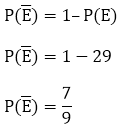(iii)  Let E = event of getting neither a white nor a black ball

No. of favourable outcomes = 18 – 6 – 4

= 8(Total balls – no. of white balls – no. of black balls)

Probability, P(E) = Number of favourable outcomes/ Total number of outcomes

P(E) = 8/18 = 4/9

29. Find the probability that a number selected at random from the numbers 1, 2, 3…. 35 is a:

(i) Prime number (ii) Multiple of 7 (iii) Multiple of 3 or 5

Solution:

Numbers from 1, 2, 3….. 35 are a total of 35.

Total no. of possible outcomes = 35

(i) Let E = event of getting a prime number

No. of favourable outcomes =11 {2, 3, 5, 7, 11, 13, 17, 19, 23, 29, 31)

Probability, P(E) = Number of favourable outcomes/ Total number of outcomes

P(E) = 11/35

(ii) Let E = event of getting a number which is a multiple of 7

No. of favourable outcomes = 5 {7, 14, 21, 28, 35}

Probability, P(E) = Number of favourable outcomes/ Total number of outcomes

P(E) = 5/35 = 1/7

(iii)  Let E = Event of getting no which is multiple of 3 or 5

No. of favourable outcomes = 16 {3, 6, 9, 12, 15, 18, 21, 24, 27, 30, 33, 5, 10, 20, 25, 35}

Probability, P(E) = Number of favourable outcomes/ Total number of outcomes

P(E) = 16/35

30. From a pack of 52 playing cards Jacks, queens, kings and aces of red colour are removed. From the remaining, a card is drawn at random. Find the probability that the card drawn is

(i) a black queen (ii) a red card

(iii) a black jack (iv) a picture card (Jacks. queens and kings are picture cards)

Solution:

We know that,

Total no. of cards = 52

All jacks, queens & kings, aces of red colour are removed.

Total no. of possible outcomes = 52 – 2 – 2 – 2 – 2 = 44 (remaining cards)

(i) Let E = event of getting a black queen

No. of favourable outcomes = 2 (queen of spade & club)

Probability, P(E) = Number of favourable outcomes/ Total number of outcomes

P(E) = 1/22

(ii)  Let E = event of getting a red card

No. of favourable outcomes = 26 – 8

= 18 (total red cards jacks – queens, kings, aces of red colour)

Probability, P(E) = Number of favourable outcomes/ Total number of outcomes

P(E) = 18/44 = 9/22

(iii)  Let E = event of getting a black jack

No. of favourable outcomes = 2 (jack of club & spade)

Probability, P(E) = Number of favourable outcomes/ Total number of outcomes

P(E) = 2/44 = 1/22

(iv) Let E = event of getting a picture card

No. of favourable outcomes = 6 (2 jacks, 2 kings & 2 queens of black colour)

Probability, P(E) = Number of favourable outcomes/ Total number of outcomes

P(E) = 6/44 = 3/22

31. A bag contains lemon flavoured candies only. Malini takes out one candy without looking into the bag. What is the probability that she takes out:

(i) an orange flavoured candy

(ii) a lemon flavoured candy

Solution:

(i)  We know that the bag contains lemon flavoured candies only. So, the event that Malini will take out an orange flavoured candy is an impossible event.

Thus, the probability of impossible event is 0

P(an orange flavoured candy) = 0

(ii)   As the bag contains lemon flavoured candies only. Then, the event that Malini will take out a lemon flavoured candy is sure event. Thus, the probability of sure event is 1.

P(a lemon flavoured candy) = 1

32. It is given that in a group of 3 students, the probability of 2 students not having the same birthday is 0.992. What is the probability that the 2 students have the same birthday?

Solution:

If E = event of 2 students not having same birthday

Given, P(E) = 0.992

Let,= event of 2 students having the same birthday.

We know that,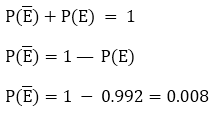Therefore, the probability that the 2 students have the same birthday is 0.008

33. A bag contains 3 red balls and 5 black balls. A ball is drawn at random from the bag. What is the probability that the ball drawn is (i) red (ii) not red

Solution:

Given,

A bag contains 3 red and 5 black balls.

So, the total no. of possible outcomes = 8 (3 red + 5 black)

(i) Let E = event of getting red ball.

No. of favourable outcomes = 3 (as there are 3 red)

Probability, P(E) = Number of favourable outcomes/ Total number of outcomes

P(E) = 3/8

(ii)  Let= event of getting no red ball.

From the previous question we already have P(E) = 3/8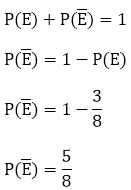34. A box contains 5 red marbles, 8 white marbles and 4 green marbles. One marble is taken out of the box at random. What is the probability that the marble taken out will be (i) red (ii) not green

Solution:

Given,

A box containing 5 red, 8 white and 4 green marbles.

So, the total no. of possible outcomes = 17 (5 red + 8 white + 4 green)

(i) Let E = Event of getting a red marble

Number of favourable outcomes = 5 (as 5 red marbles)

Probability, P(E) = Number of favourable outcomes/ Total number of outcomes

P(E) = 5/17

(ii) Let E= event of getting a green marble

Number of favourable outcomes = 4 (as 4 green marbles)

Probability, P(E) = Number of favourable outcomes/ Total number of outcomes

P(E) = 4/17

So,= Event of getting not a green marble

Then we know that,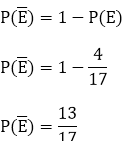Therefore, the probability that the marble taken out is not green is 13/17.

35. A lot consists of 144 ball pens of which 20 are defective and others good. Nuri will buy a pen if it is good, but will not buy if it is defective. The shopkeeper draws one pen at random and gives it to her. What is the probability that

Solution:

No. of good pens = 144 – 20 = 124

No. of detective pens = 20

Total no. of possible outcomes =144 (total no. of pens)

(i) For her to buy it the pen should be a good one.

So, let E = event of buying a pen which is good.

No. of favourable outcomes = 124 (124 good pens)

Probability, P(E) = Number of favourable outcomes/ Total number of outcomes

P(E) = 124/144 = 31/36

(ii)  Now, Let= Event of she not buying a pen as it was a defective one.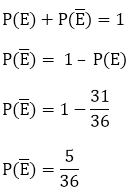Therefore, the probability that she will not buy = 5/36

36. 12 defective pens are accidently mixed with 132 good ones. It is not possible to just look at pen and tell whether or not it is defective. One pen is taken out at random from this lot. Determine the probability that the pen taken out is good one.

Solution:

We have,

No. of good pens = 132

No. of defective pens = 12

So, the total no. of pens = 132 + 12 = 144

Then, the total no. of possible outcomes = 144

Now, let E = event of getting a good pen.

No. of favourable out comes = 132 {132 good pens}

Probability, P(E) = Number of favourable outcomes/ Total number of outcomes

P(E) = 132/144 = 11/12